# Simple harmonic motion Questions and Answers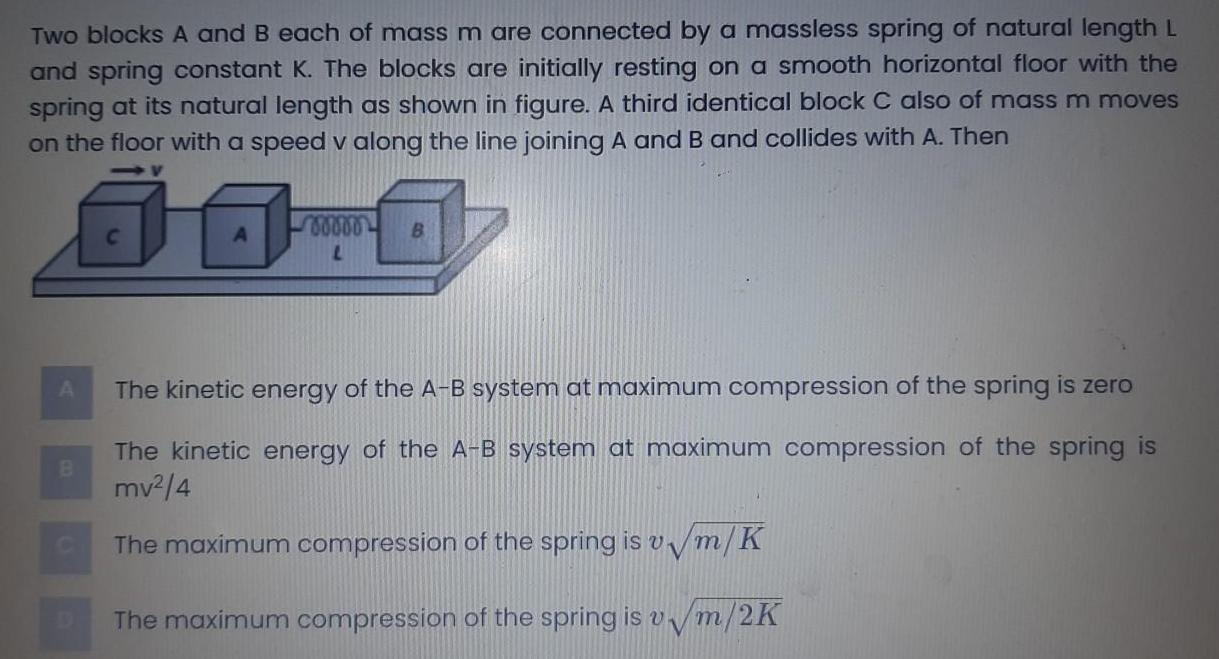Physics
Simple harmonic motion
Two blocks A and B each of mass m are connected by a massless spring of natural length L and spring constant K The blocks are initially resting on a smooth horizontal floor with the spring at its natural length as shown in figure A third identical block C also of mass m moves on the floor with a speed v along the line joining A and B and collides with A Then A B C 00000 L B The kinetic energy of the A B system at maximum compression of the spring is zero The kinetic energy of the A B system at maximum compression of the spring is mv 4 The maximum compression of the spring is v m K The maximum compression of the spring is v m 2K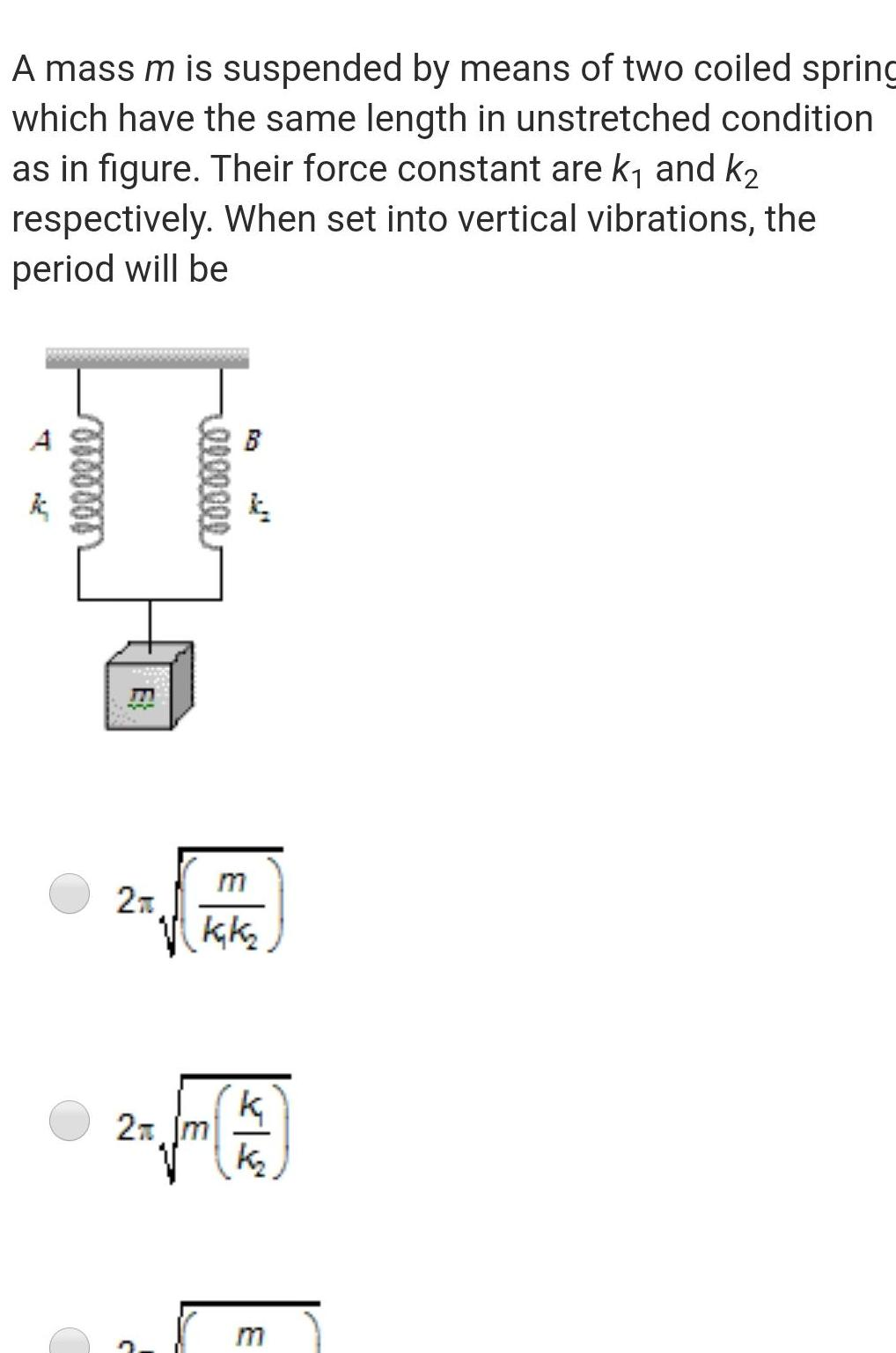Physics
Simple harmonic motion
A mass m is suspended by means of two coiled spring which have the same length in unstretched condition as in figure Their force constant are k and k respectively When set into vertical vibrations the period will be T 0000004 E 2T 0000000 A m kak 2 Jm k K 3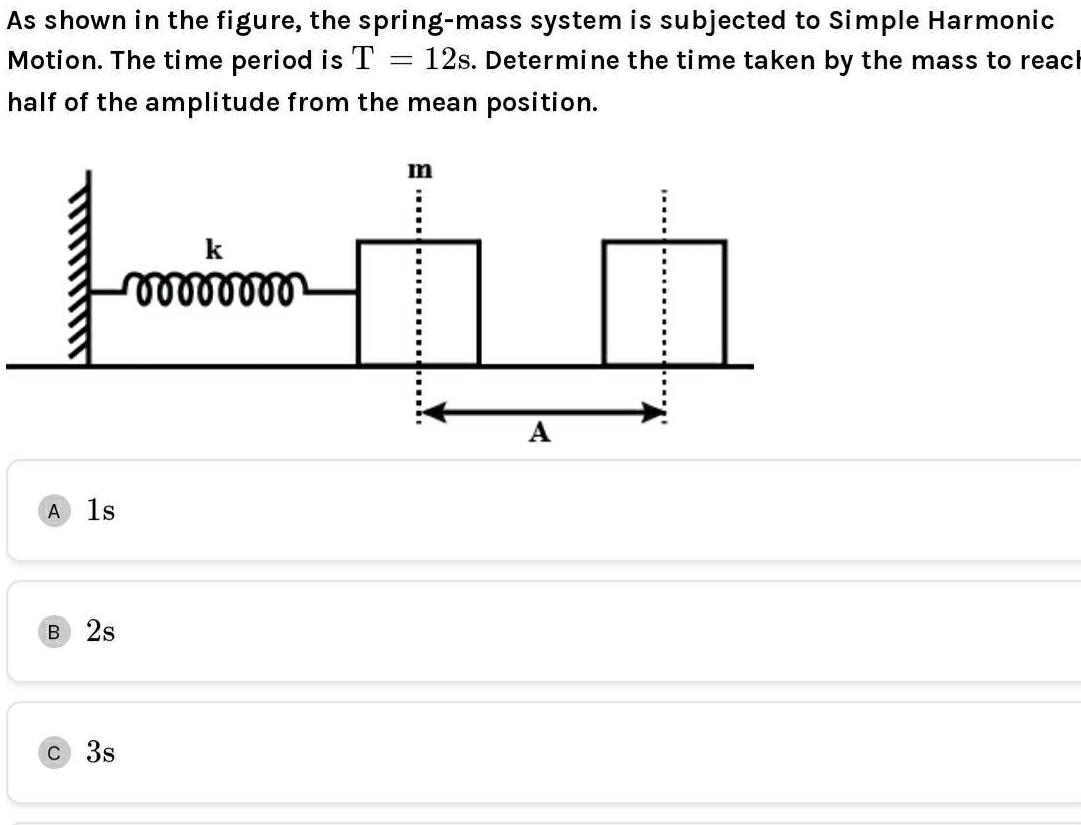Physics
Simple harmonic motion
As shown in the figure the spring mass system is subjected to Simple Harmonic 12s Determine the time taken by the mass to reach half of the amplitude from the mean position Motion The time period is T A 1s B 2s C 3s k mmmmmmm m APhysics
Simple harmonic motion
A particle of mass m is attached to a spring of spring constant k and has a natural angular frequency 0 An external force F t proportional to cosot w w0 is applied to the oscillator The time displacement of the oscillator will be proportional to A B m 1 m w w 1 m w w m w w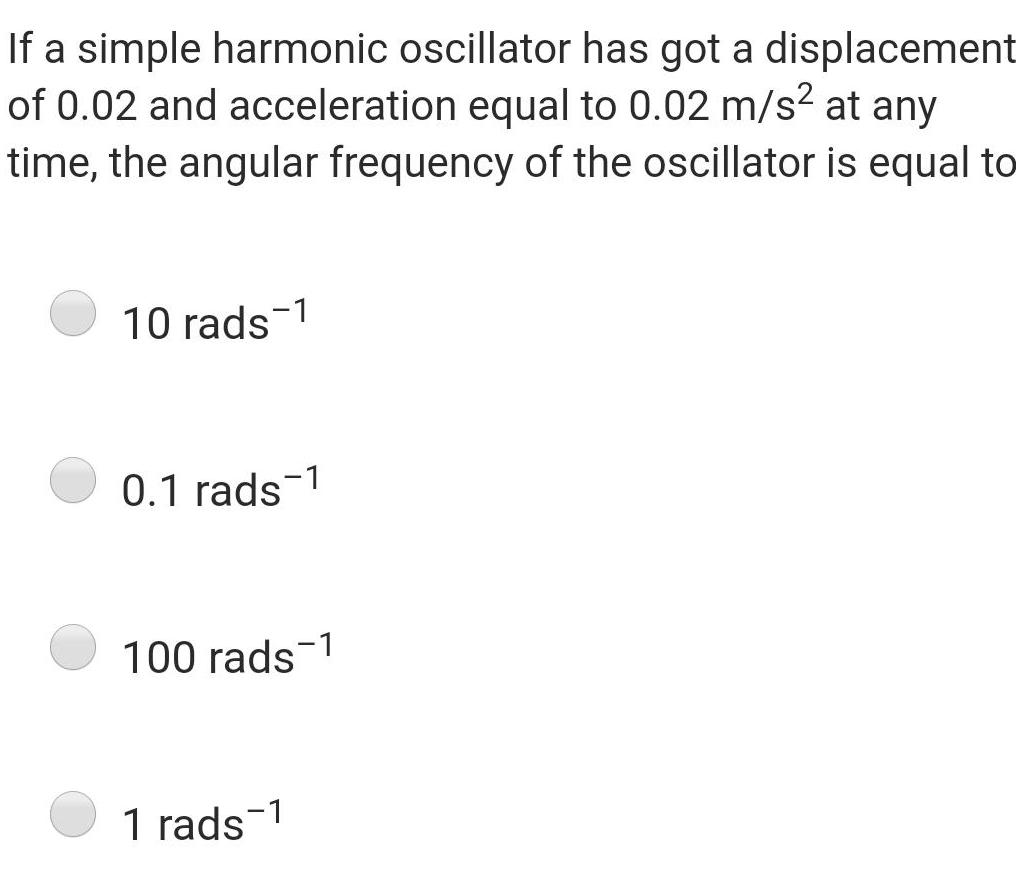Physics
Simple harmonic motion
If a simple harmonic oscillator has got a displacement of 0 02 and acceleration equal to 0 02 m s at any time the angular frequency of the oscillator is equal to 10 rads 1 0 1 rads 1 100 rads 1 1 rads 1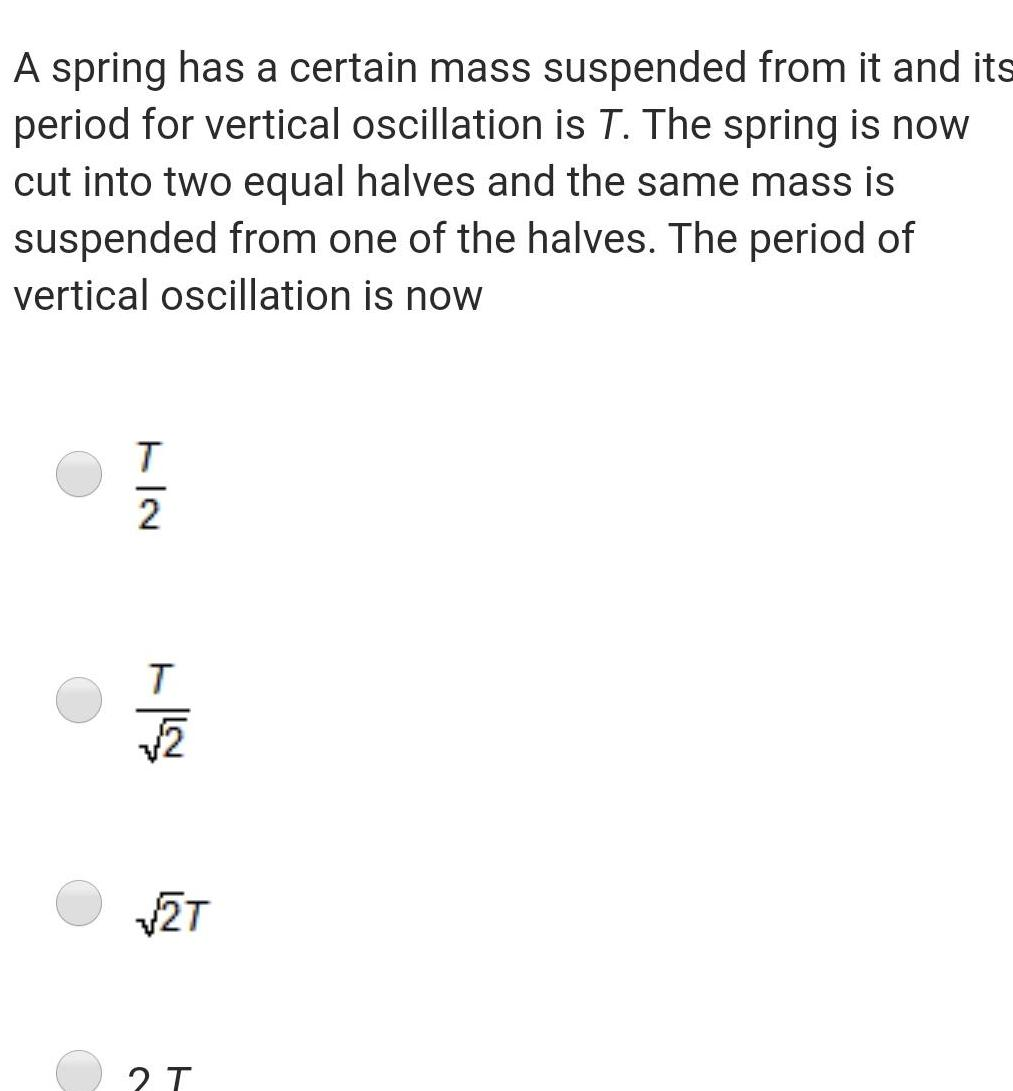Physics
Simple harmonic motion
A spring has a certain mass suspended from it and its period for vertical oscillation is T The spring is now cut into two equal halves and the same mass is suspended from one of the halves The period of vertical oscillation is now 7 2 T T 251 2T 2 T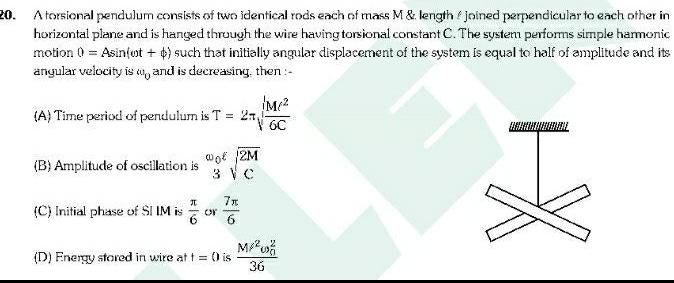Physics
Simple harmonic motion
20 A torsional pendulum consists of two identical rods each of mass M length joined perpendicular to each other in horizontal plane and is hanged through the wire having torsional constant C The system performs simple harmonic motion 0 Asinfot such that initially angular displacement of the system is equal to half of amplitude and its angular velocity is w and is decreasing then A Time period of pendulum is T B Amplitude of oscillation is J C Initial phase of SI IM is IM 26C wo 12M 3 V C 7x D Energy stored in wire at t 0 is M 36 ENDIL I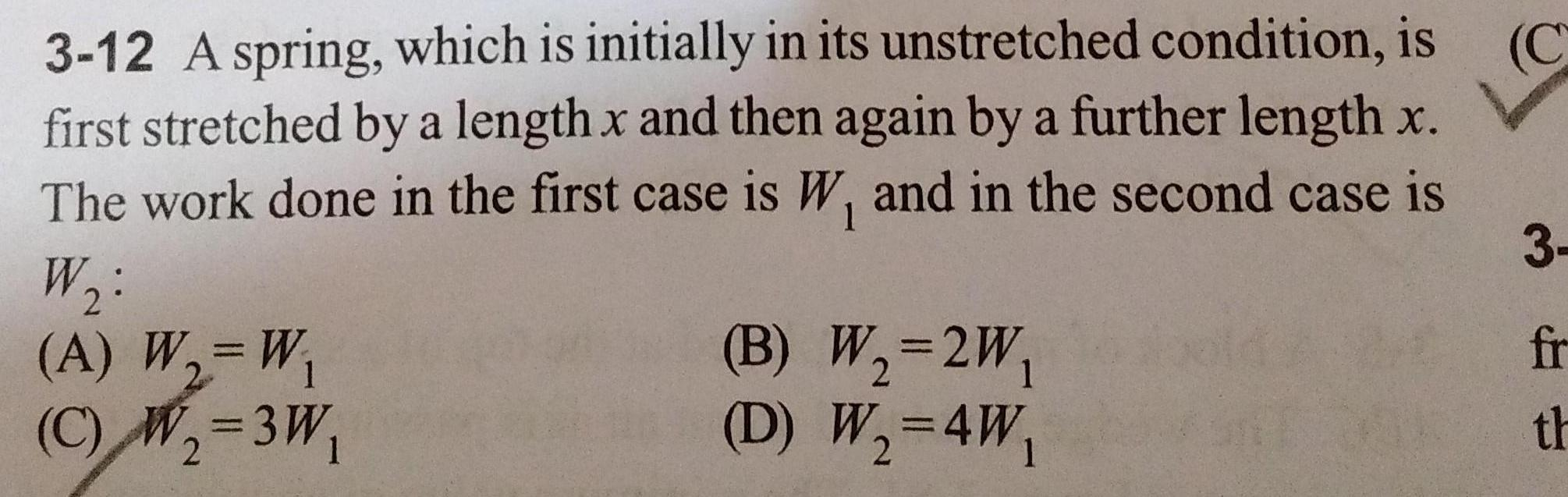Physics
Simple harmonic motion
C 3 12 A spring which is initially in its unstretched condition is first stretched by a length x and then again by a further length x The work done in the first case is W and in the second case is W A W W 2 1 C W 3W 2 B W 2W D W 4W 3 fr th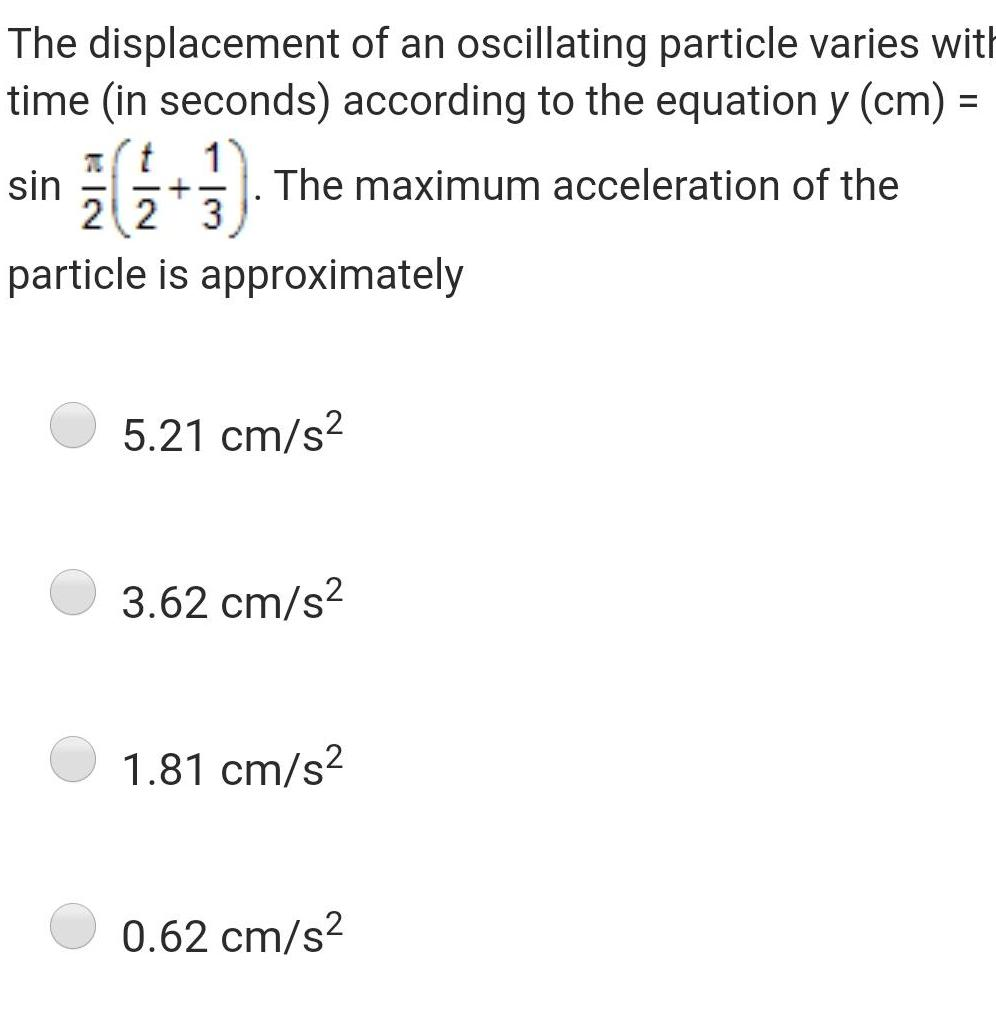Physics
Simple harmonic motion
The displacement of an oscillating particle varies with time in seconds according to the equation y cm sin 70 t 1 The maximum acceleration of the 22 3 particle is approximately 5 21 cm s 3 62 cm s 1 81 cm s 0 62 cm sPhysics
Simple harmonic motion
A tunnel has been dug through the centre of the earth and a ball is released in it It will reach the other end of the tunnel after 84 6 minutes 42 3 minutes 1 day Will not reach the other end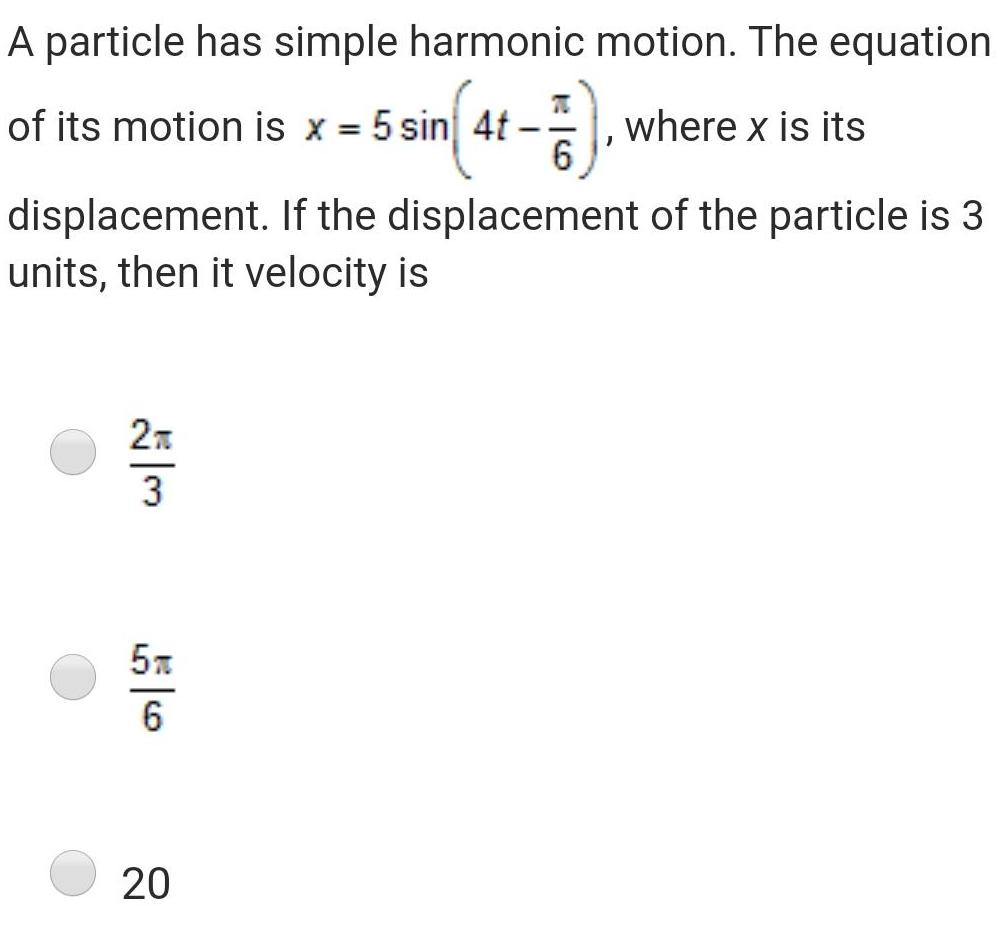Physics
Simple harmonic motion
A particle has simple harmonic motion The equation sin 4 7 where x is its 6 of its motion is x 5 sin 4t displacement If the displacement of the particle is 3 units then it velocity is 2T 3 5m 6 20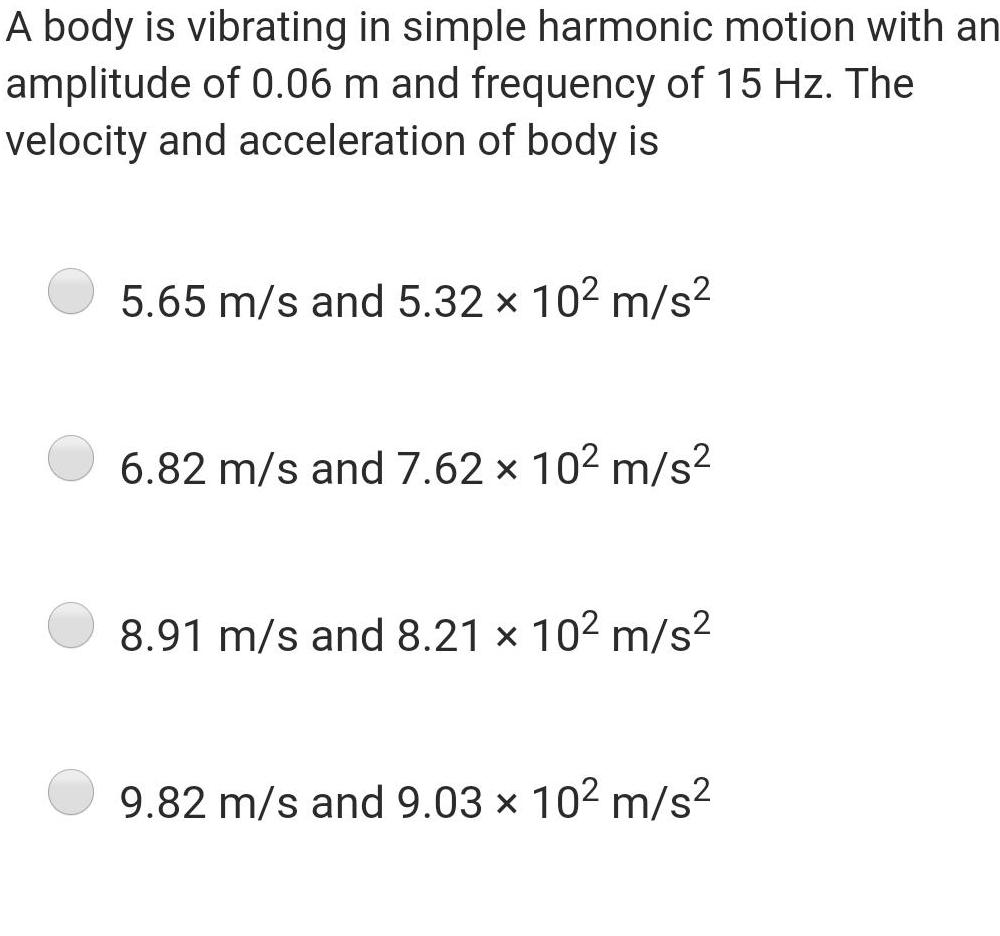Physics
Simple harmonic motion
A body is vibrating in simple harmonic motion with an amplitude of 0 06 m and frequency of 15 Hz The velocity and acceleration of body is 5 65 m s and 5 32 10 m s X 6 82 m s and 7 62 x 10 m s 8 91 m s and 8 21 x 10 m s 9 82 m s and 9 03 10 m s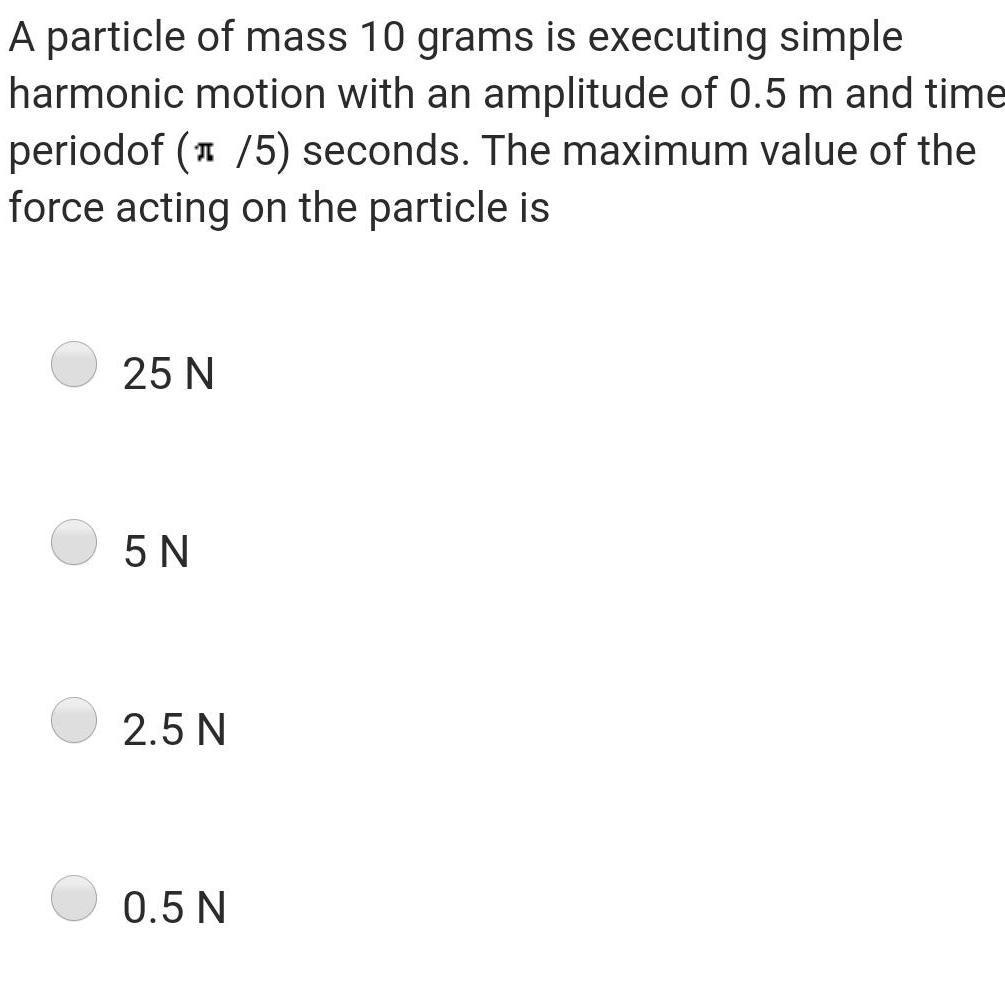Physics
Simple harmonic motion
A particle of mass 10 grams is executing simple harmonic motion with an amplitude of 0 5 m and time periodof 1 5 seconds The maximum value of the force acting on the particle is 25 N 5 N 2 5 N 0 5 N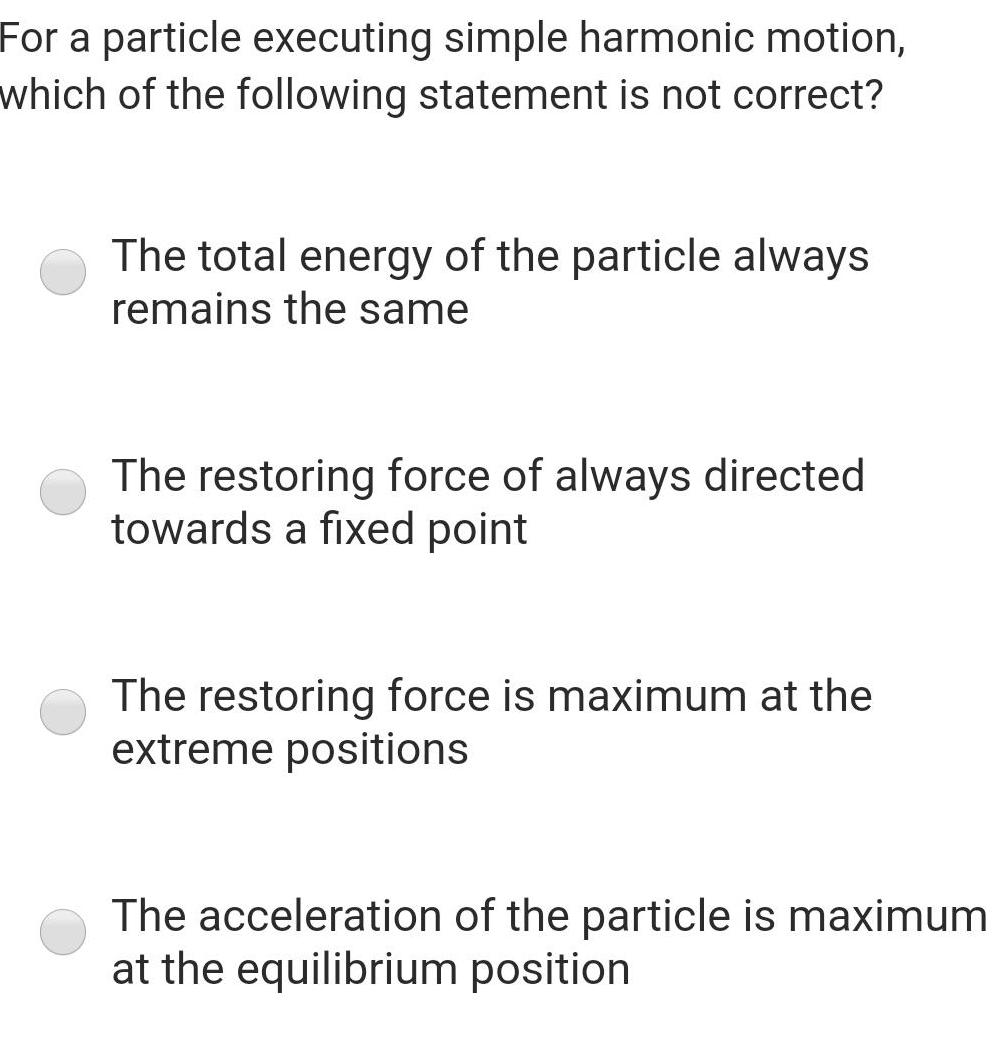Physics
Simple harmonic motion
For a particle executing simple harmonic motion which of the following statement is not correct The total energy of the particle always remains the same The restoring force of always directed towards a fixed point The restoring force is maximum at the extreme positions The acceleration of the particle is maximum at the equilibrium position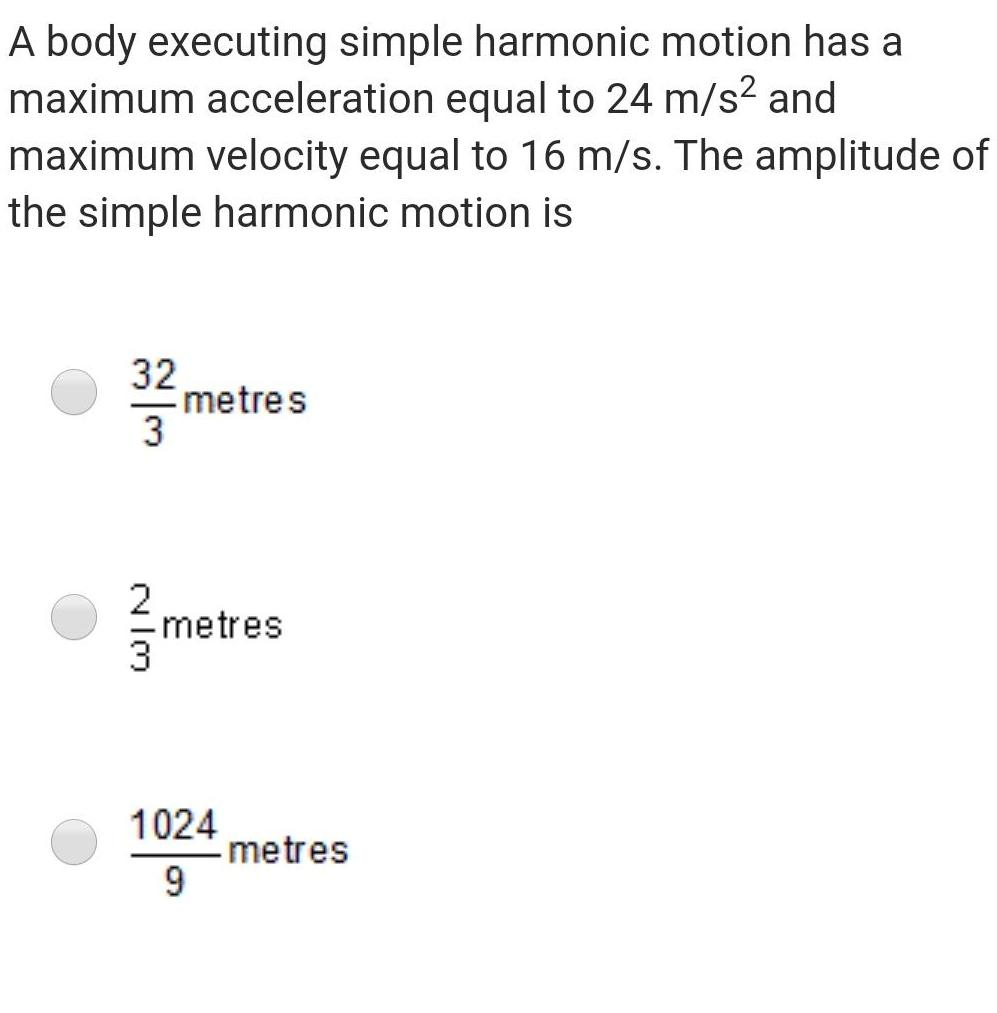Physics
Simple harmonic motion
A body executing simple harmonic motion has a maximum acceleration equal to 24 m s and maximum velocity equal to 16 m s The amplitude of the simple harmonic motion is 32 3333 2 metres metres 1024 9 metres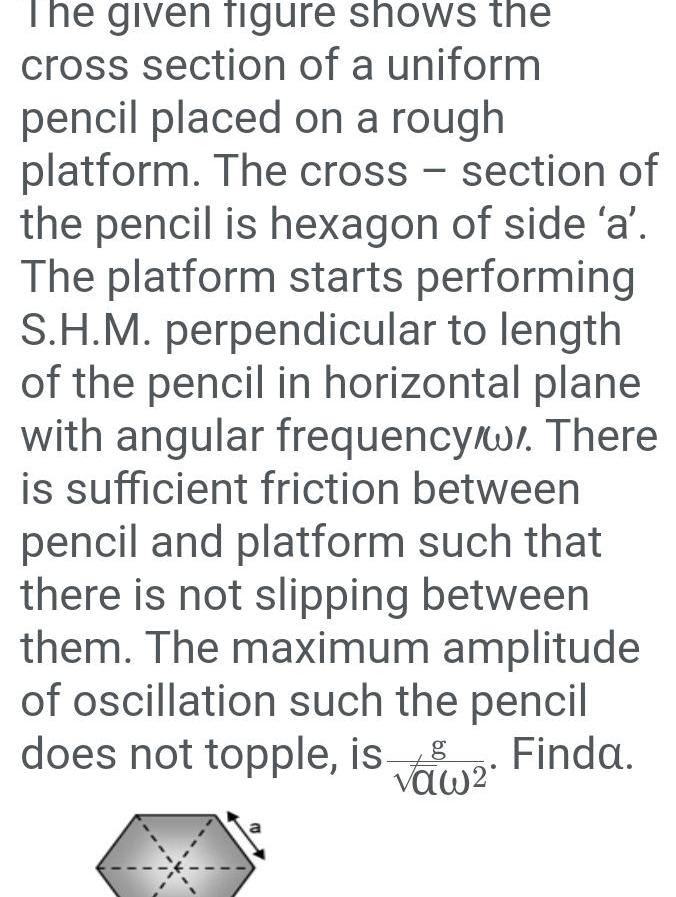Physics
Simple harmonic motion
The given figure shows the cross section of a uniform pencil placed on a rough platform The cross section of the pencil is hexagon of side a The platform starts performing S H M perpendicular to length of the pencil in horizontal plane with angular frequency w There is sufficient friction between pencil and platform such that there is not slipping between them The maximum amplitude of oscillation such the pencil does not topple is 2 Finda g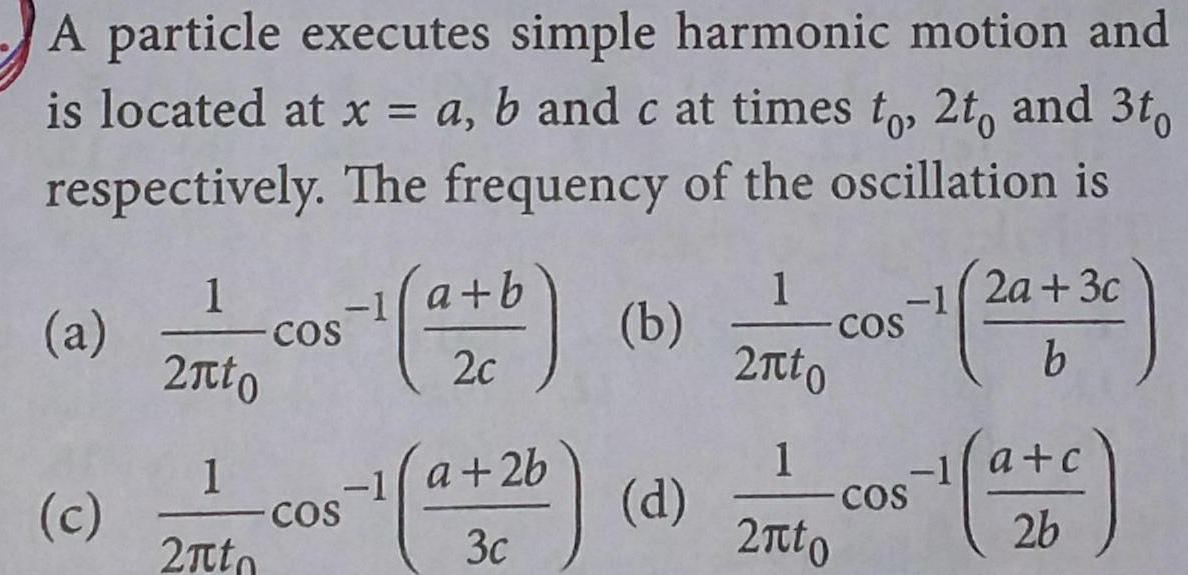Physics
Simple harmonic motion
A particle executes simple harmonic motion and is located at x a b and c at times to 2t and 3to respectively The frequency of the oscillation is a c 1 2 to 1 2 to 1 COS COS a b 2c 1 a 2b 3c b d 1 2 to 1 2 to COS COS 2a 3c b 26Physics
Simple harmonic motion
d Both assertion and reason are false 17 A particle is executing linear simple harmonic motion The fraction of the total energy that c Assertion is true but reason is false is not the correct explanation of the assertion b Both assertion and reason are true but reason AIIMS and 16 TC time in second maga ely P of of a sufficie to and fro he acceleration ns 2 The time pe harmonic mot the partide magnitude of leration Then d nonic motion displacement respective yi 42 be c K 2K JEE Main 13 The mass and the diameter of a planet are three times the respective values for the Earth The period of oscillation of a simple pendulum on the Earth is 2 s The period of oscillation of the same pendulum on the planet would be 3 a 2 3s b s 2 d K c a 1 5 Hz c 3 5 Hz 2 2 K 4 S d 3 b 2 5 Hz d 4 5 Hz 2 JEE Main A simple pendulum of length 1 m is oscillating with an angular frequency 10 rad s The support of the pendulum starts oscillating up and down with a small angular frequency of 1 rad s and an amplitude of 10 2 m The relative change in the angular frequency of the pendulum is best given by a 10 5 rad s c 1 rad s b 10 rad s d 10 rad s S JEE Main 15 A bottle weighing 220 g and of area of cross section 50 cm and height 4 cm oscillates on the surface of water in vertical position Its frequency of oscillation is AIIMS 16 Assertion The periodic time of a hard spring i less as compared to that of a soft spring Reason The periodic time depends upon th spring constant which is large for hard spring a Both assertion and reason are true and reaso is the correct explanation of the assertion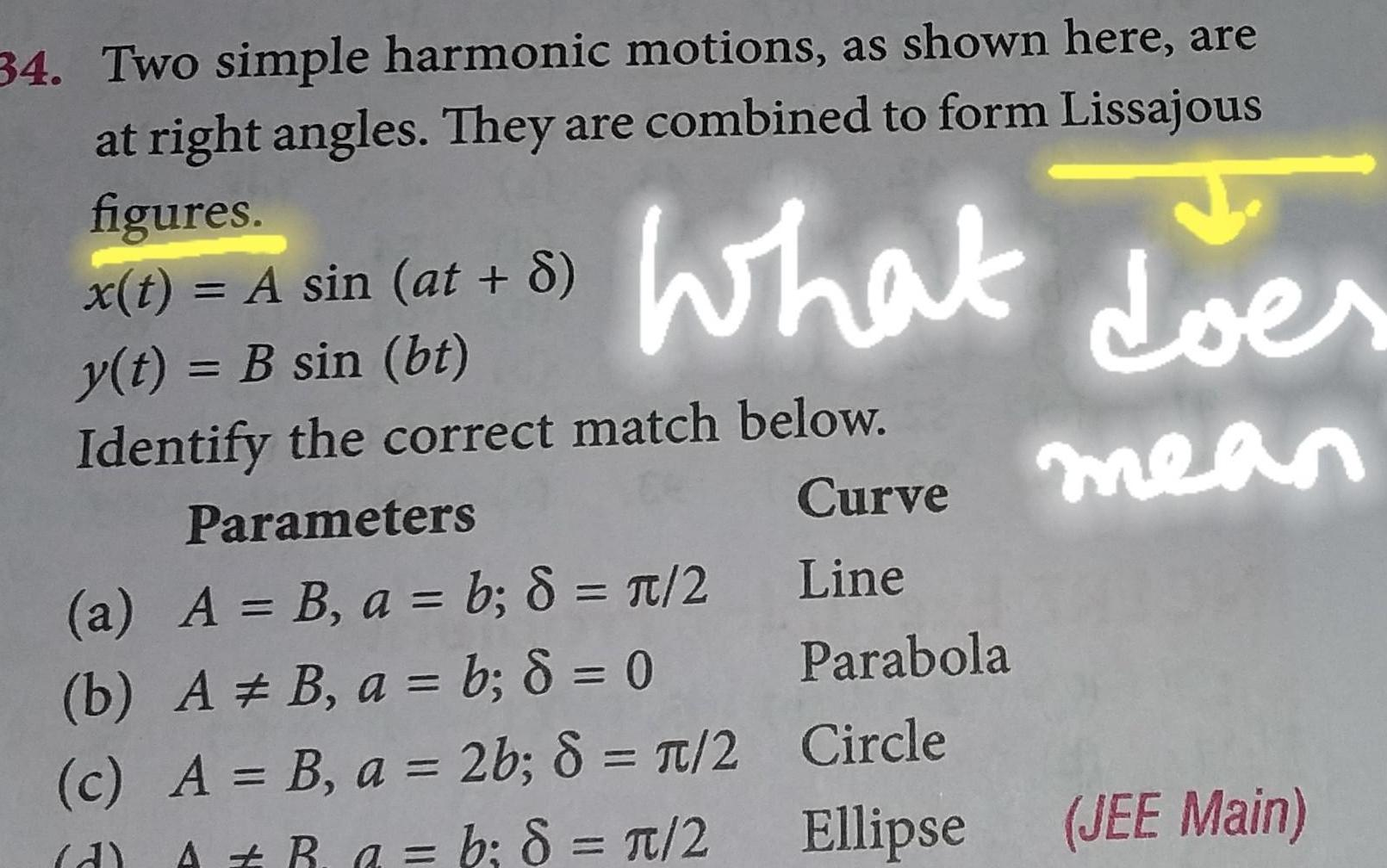Physics
Simple harmonic motion
34 Two simple harmonic motions as shown here are at right angles They are combined to form Lissajous What doe figures x t A sin at 8 y t B sin bt Identify the correct match below Parameters a A B a b 8 2 b A B a b 8 0 c A B a 2b 8 2 A R a b 8 2 d Curve mean Line Parabola Circle Ellipse JEE Main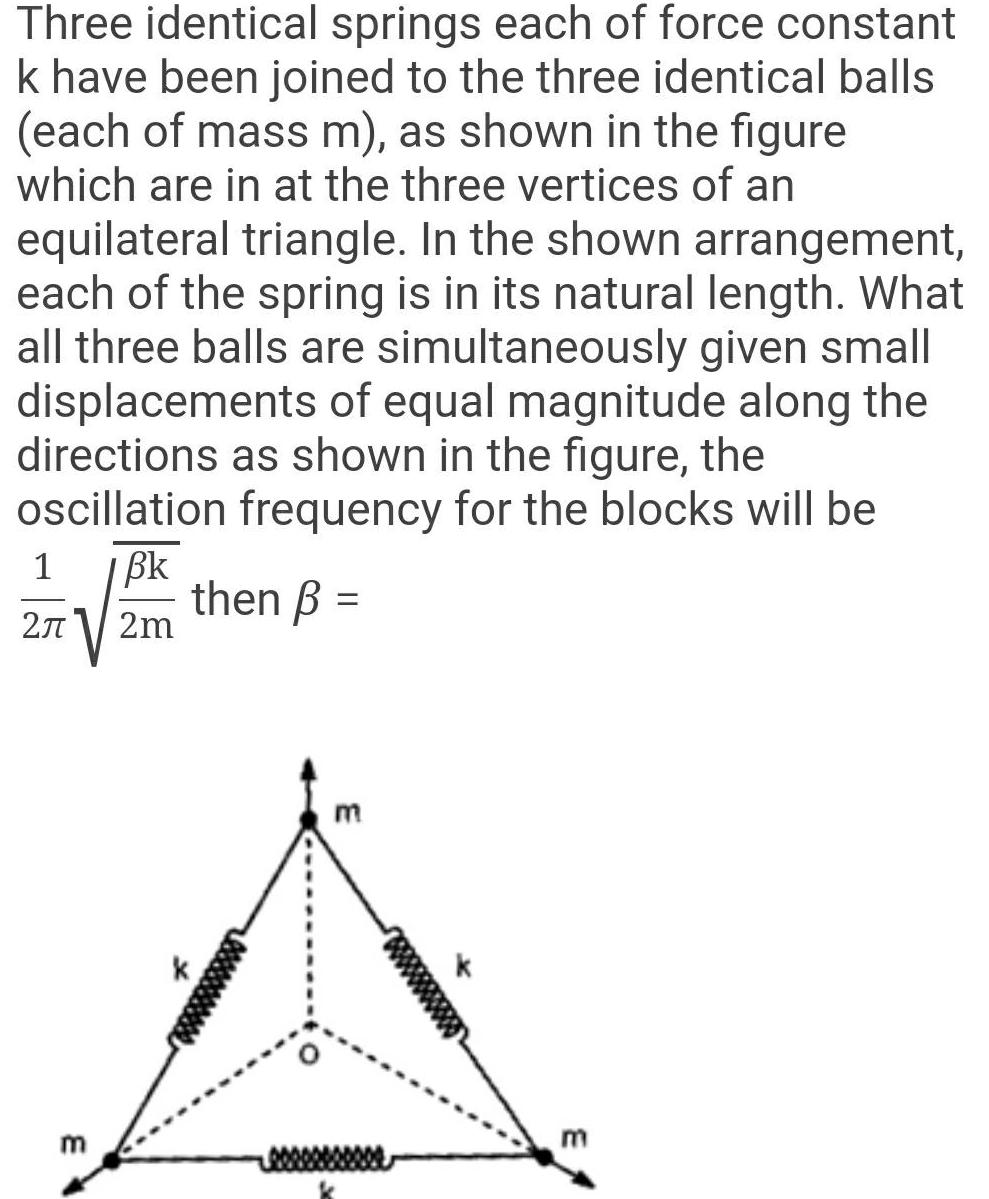Physics
Simple harmonic motion
Three identical springs each of force constant k have been joined to the three identical balls each of mass m as shown in the figure which are in at the three vertices of an equilateral triangle In the shown arrangement each of the spring is in its natural length What all three balls are simultaneously given small displacements of equal magnitude along the directions as shown in the figure the oscillation frequency for the blocks will be Bk then 2m 1 2 m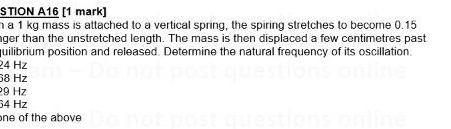Physics
Simple harmonic motion
STION A16 1 mark na 1 kg mass is attached to a vertical spring the spiring stretches to become 0 15 nger than the unstretched length The mass is then displaced a few centimetres past quilibrium position and released Determine the natural frequency of its oscillation 24 Hz 38 Hz P9 Hz 34 Hz one of the above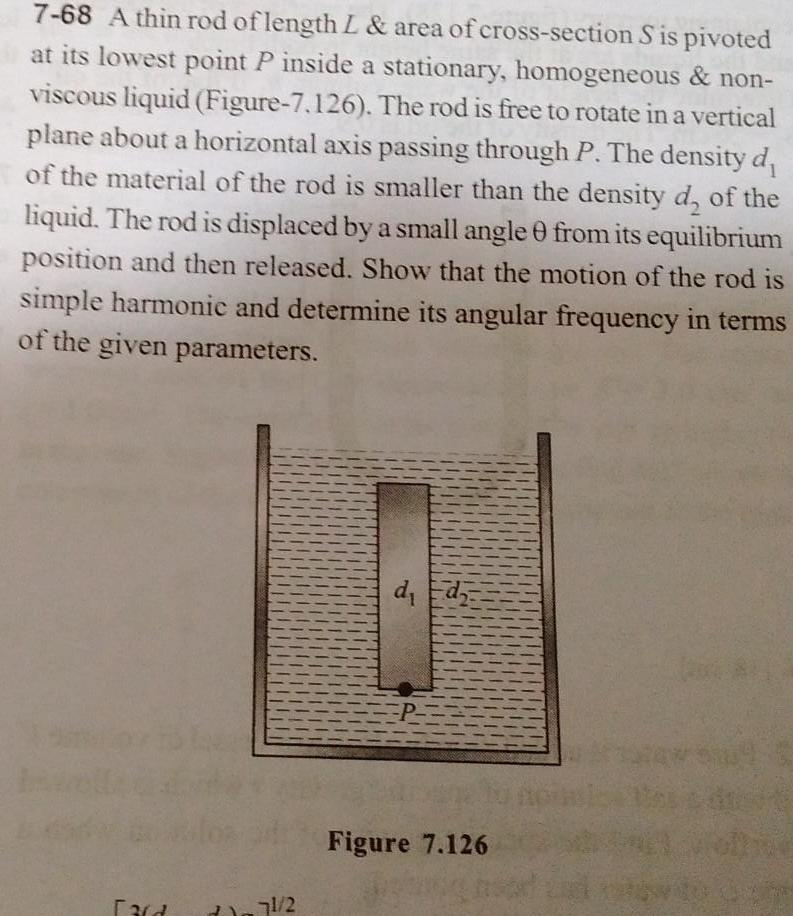Physics
Simple harmonic motion
7 68 A thin rod of length L area of cross section S is pivoted at its lowest point P inside a stationary homogeneous non viscous liquid Figure 7 126 The rod is free to rotate in a vertical plane about a horizontal axis passing through P The density d of the material of the rod is smaller than the density d of the liquid The rod is displaced by a small angle 0 from its equilibrium position and then released Show that the motion of the rod is simple harmonic and determine its angular frequency in terms of the given parameters Fard 71 2 d d Figure 7 126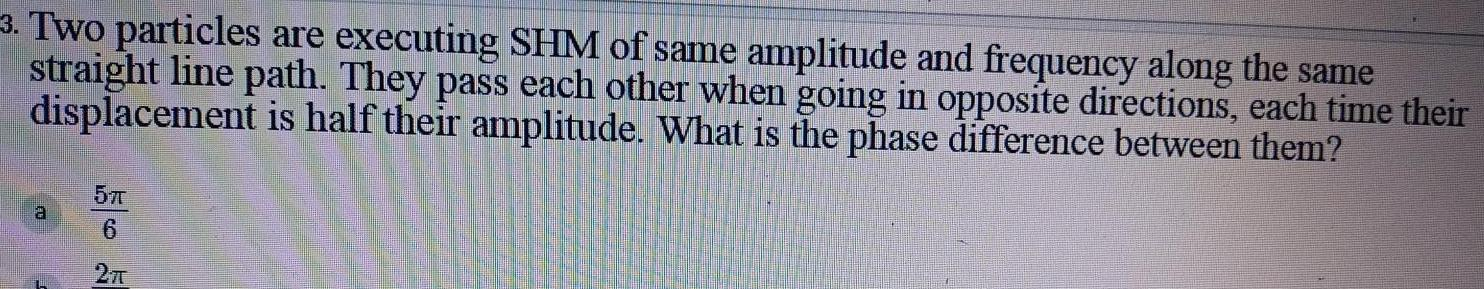Physics
Simple harmonic motion
3 Two particles are executing SHM of same amplitude and frequency along the same straight line path They pass each other when going in opposite directions each time their displacement is half their amplitude What is the phase difference between them 5T 6 2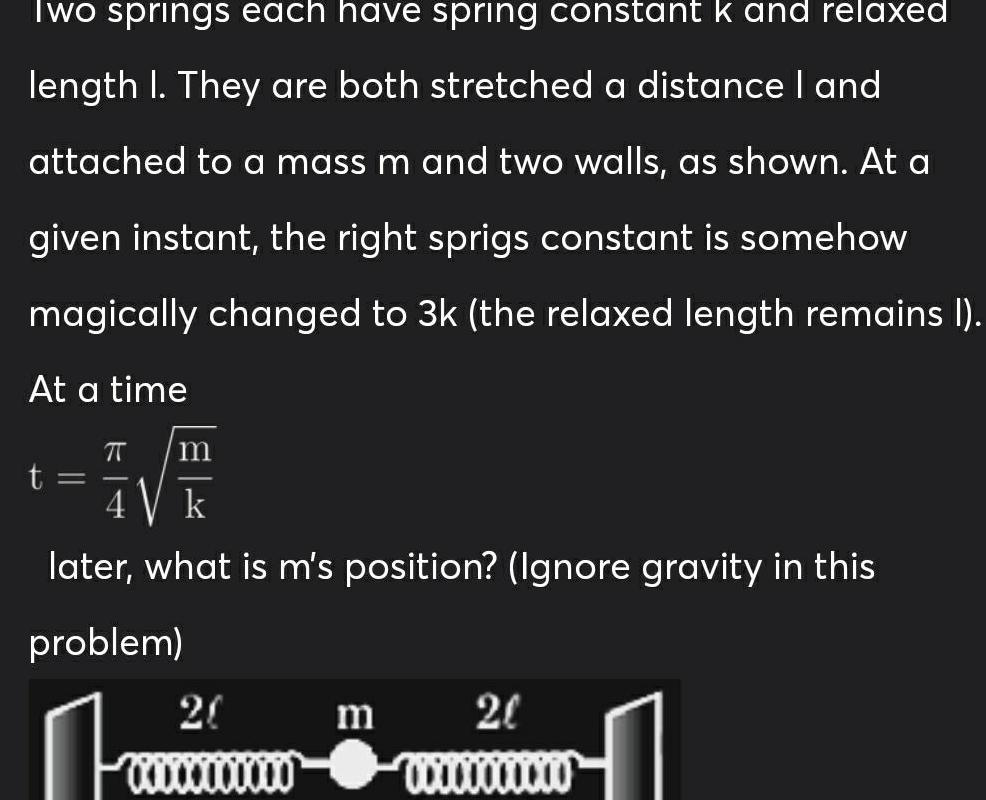Physics
Simple harmonic motion
Two springs each have spring constant k and relaxed length I They are both stretched a distance I and attached to a mass m and two walls as shown At a given instant the right sprigs constant is somehow magically changed to 3k the relaxed length remains I At a time m k later what is m s position Ignore gravity in this T problem n 20 00000000000 m 20 00000000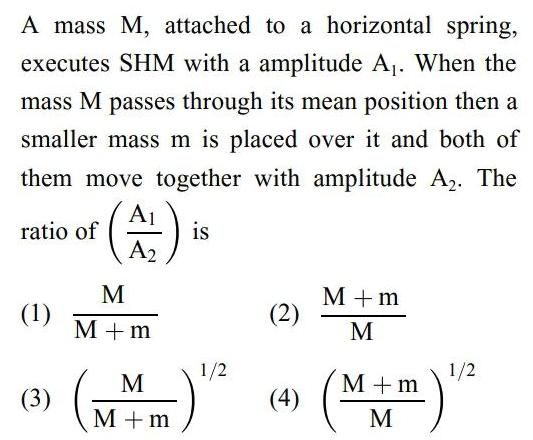Physics
Simple harmonic motion
A mass M attached to a horizontal spring executes SHM with a amplitude A When the mass M passes through its mean position then a smaller mass m is placed over it and both of them move together with amplitude A The A A2 ratio of 1 3 M M m M M m is 1 2 2 4 M m M M m M 1 2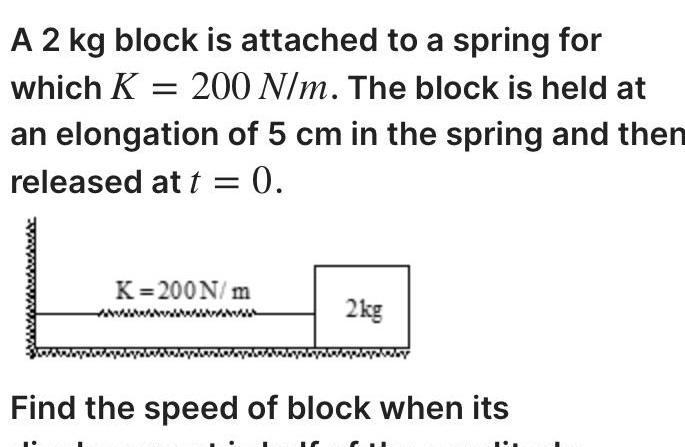Physics
Simple harmonic motion
A 2 kg block is attached to a spring for which K 200 N m The block is held at an elongation of 5 cm in the spring and then released at t 0 K 200N m 2kg Find the speed of block when its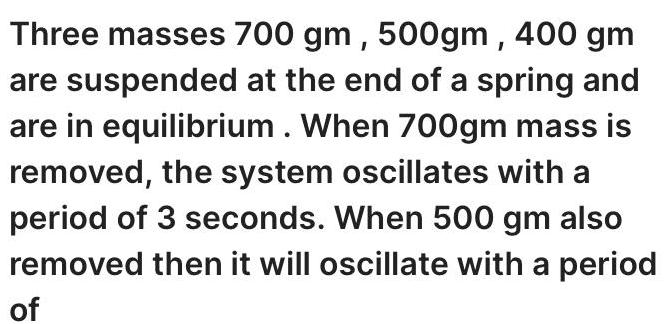Physics
Simple harmonic motion
Three masses 700 gm 500gm 400 gm are suspended at the end of a spring and are in equilibrium When 700gm mass is removed the system oscillates with a period of 3 seconds When 500 gm also removed then it will oscillate with a period of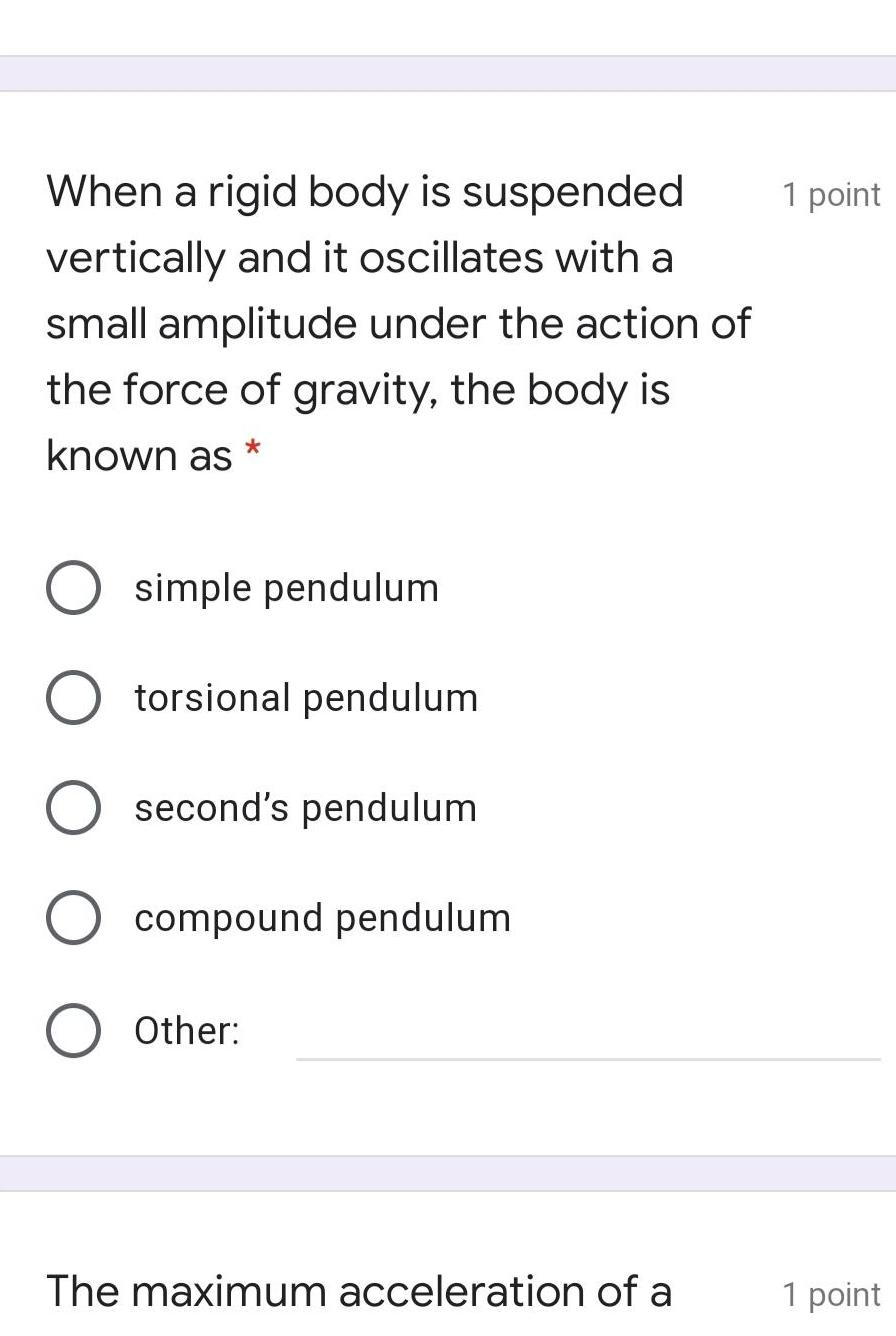Physics
Simple harmonic motion
When a rigid body is suspended vertically and it oscillates with a small amplitude under the action of the force of gravity the body is known as O simple pendulum O torsional pendulum O second s pendulum O compound pendulum O Other The maximum acceleration of a 1 point 1 point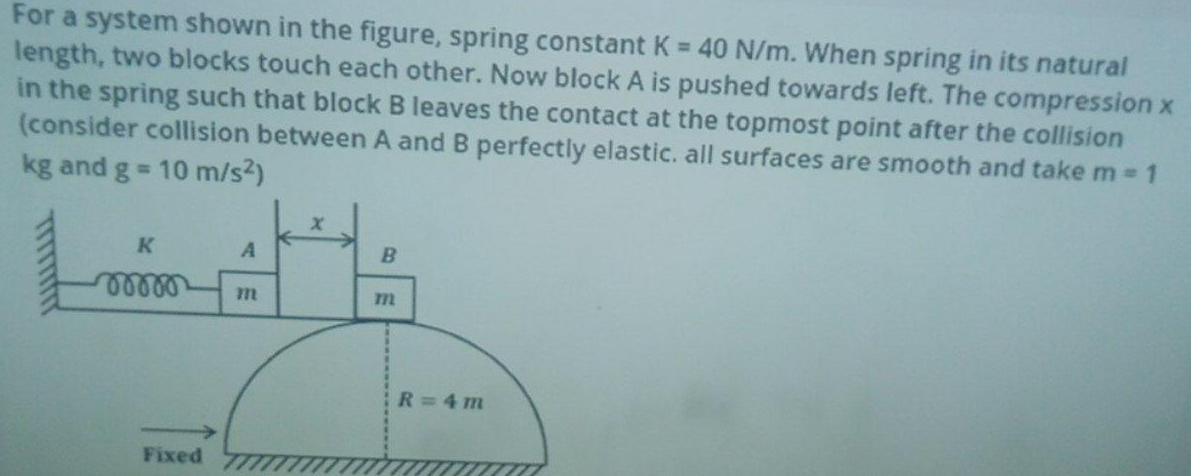Physics
Simple harmonic motion
For a system shown in the figure spring constant K 40 N m When spring in its natural length two blocks touch each other Now block A is pushed towards left The compression x in the spring such that block B leaves the contact at the topmost point after the collision consider collision between A and B perfectly elastic all surfaces are smooth and take m 1 kg and g 10 m s ALILLI K A moooo m Fixed X B m R 4m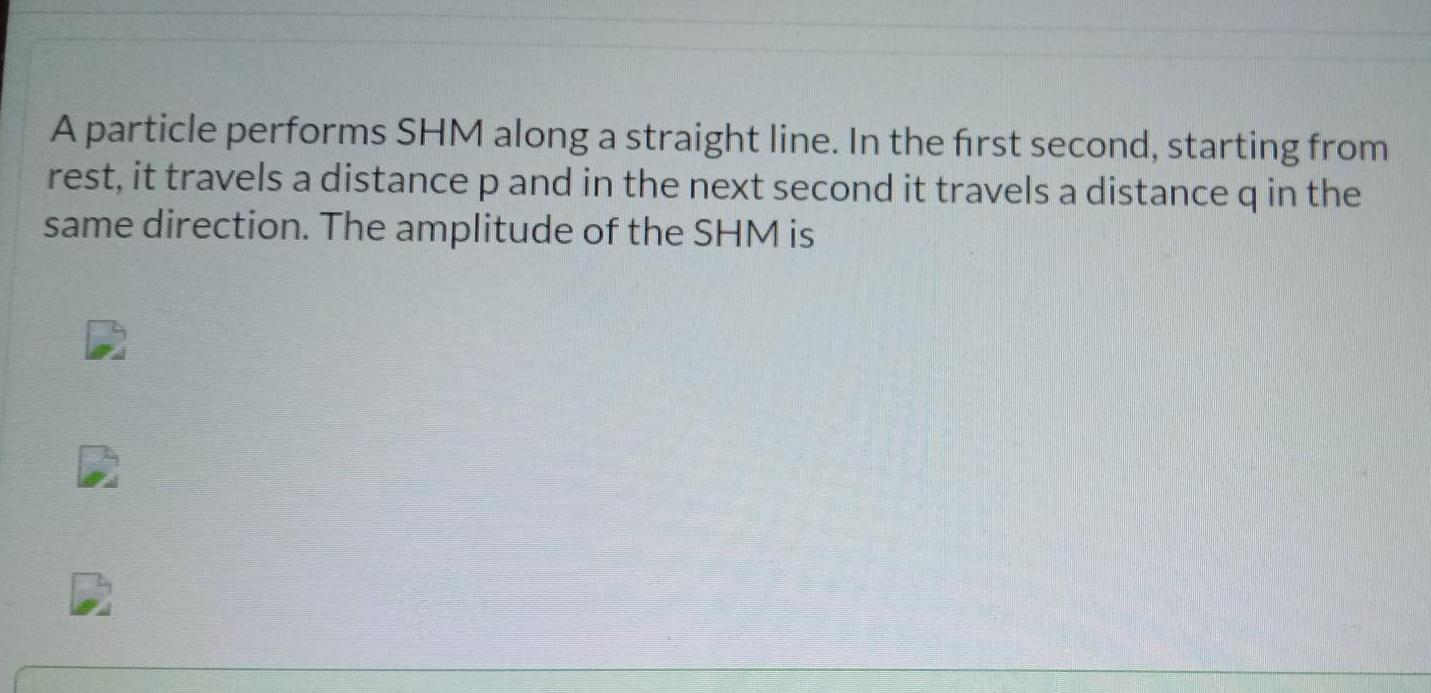Physics
Simple harmonic motion
A particle performs SHM along a straight line In the first second starting from rest it travels a distance p and in the next second it travels a distance q in the same direction The amplitude of the SHM is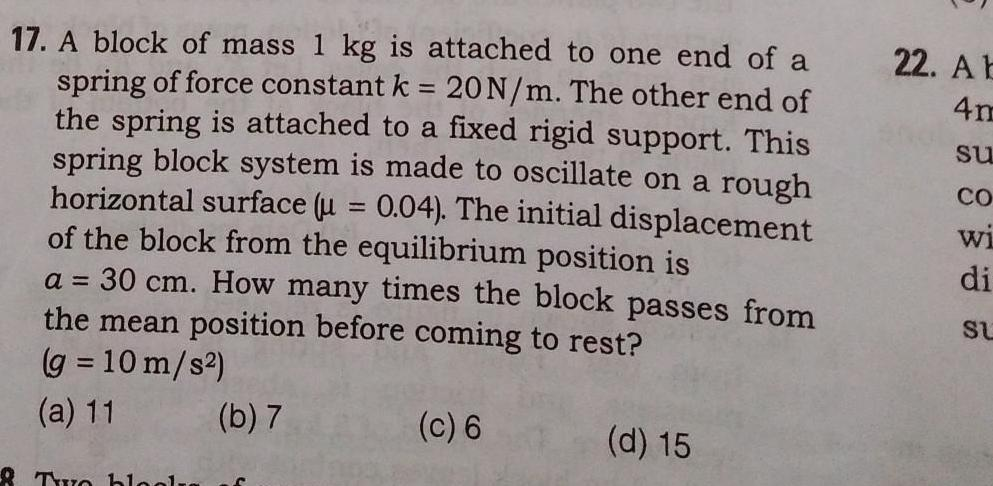Physics
Simple harmonic motion
17 A block of mass 1 kg is attached to one end of a spring of force constant k 20 N m The other end of the spring is attached to a fixed rigid support This spring block system is made to oscillate on a rough horizontal surface u 0 04 The initial displacement of the block from the equilibrium position is 1 a 30 cm How many times the block passes from the mean position before coming to rest g 10 m s a 11 c 6 The bl b 7 d 15 22 A b 4m su CO WI di SU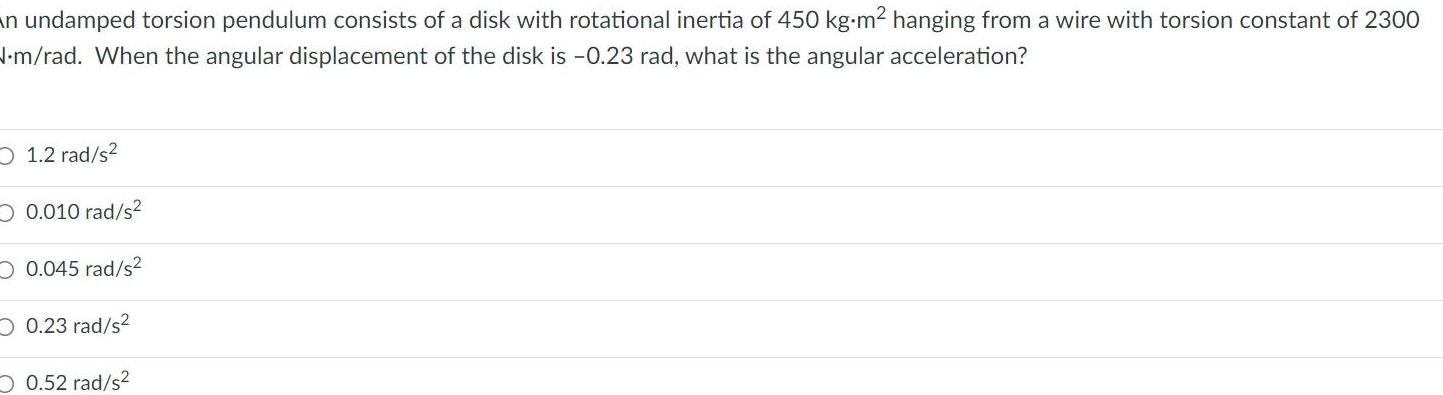Physics
Simple harmonic motion
an undamped torsion pendulum consists of a disk with rotational inertia of 450 kg m hanging from a wire with torsion constant of 2300 N m rad When the angular displacement of the disk is 0 23 rad what is the angular acceleration 1 2 rad s 0 010 rad s 0 045 rad s 0 23 rad s 0 52 rad s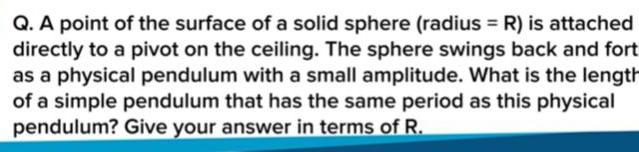Physics
Simple harmonic motion
Q A point of the surface of a solid sphere radius R is attached directly to a pivot on the ceiling The sphere swings back and fort as a physical pendulum with a small amplitude What is the length of a simple pendulum that has the same period as this physical pendulum Give your answer in terms of R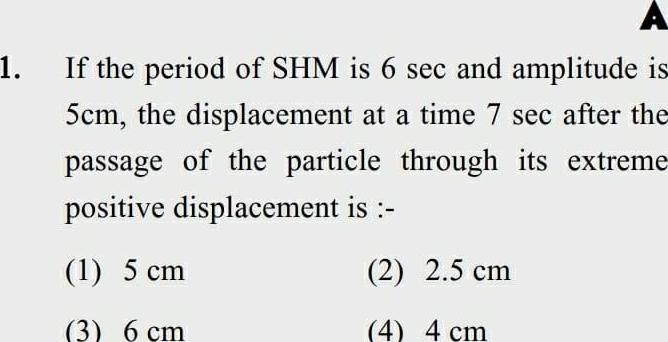Physics
Simple harmonic motion
1 A If the period of SHM is 6 sec and amplitude is 5cm the displacement at a time 7 sec after the passage of the particle through its extreme positive displacement is 1 5 cm 2 2 5 cm 3 6 cm 4 4 cm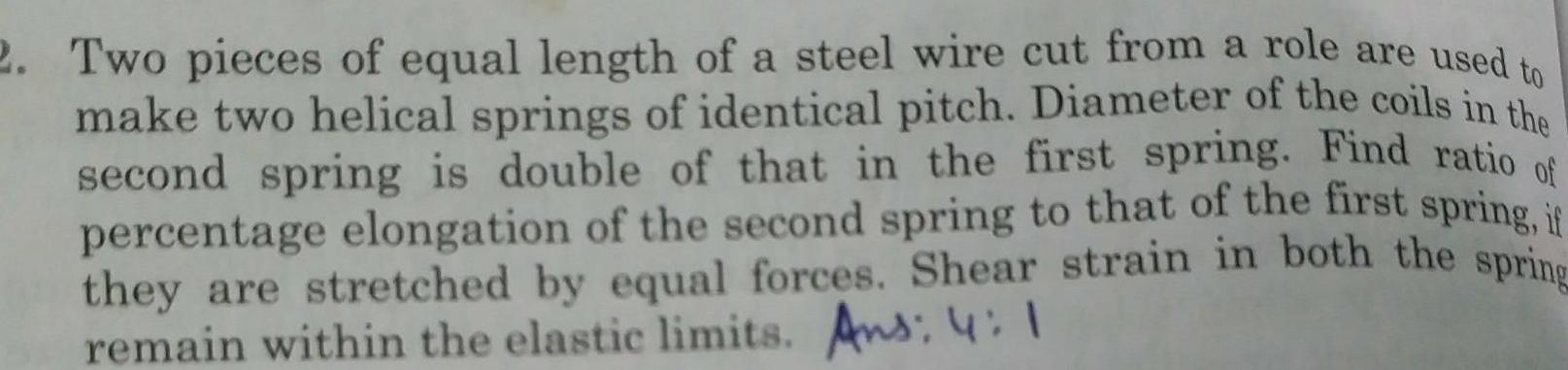Physics
Simple harmonic motion
of 2 Two pieces of equal length of a steel wire cut from a role are used to make two helical springs of identical pitch Diameter of the coils in the second spring is double of that in the first spring Find ratio percentage elongation of the second spring to that of the first spring if they are stretched by equal forces Shear strain in both the spring remain within the elastic limits Ans 4 1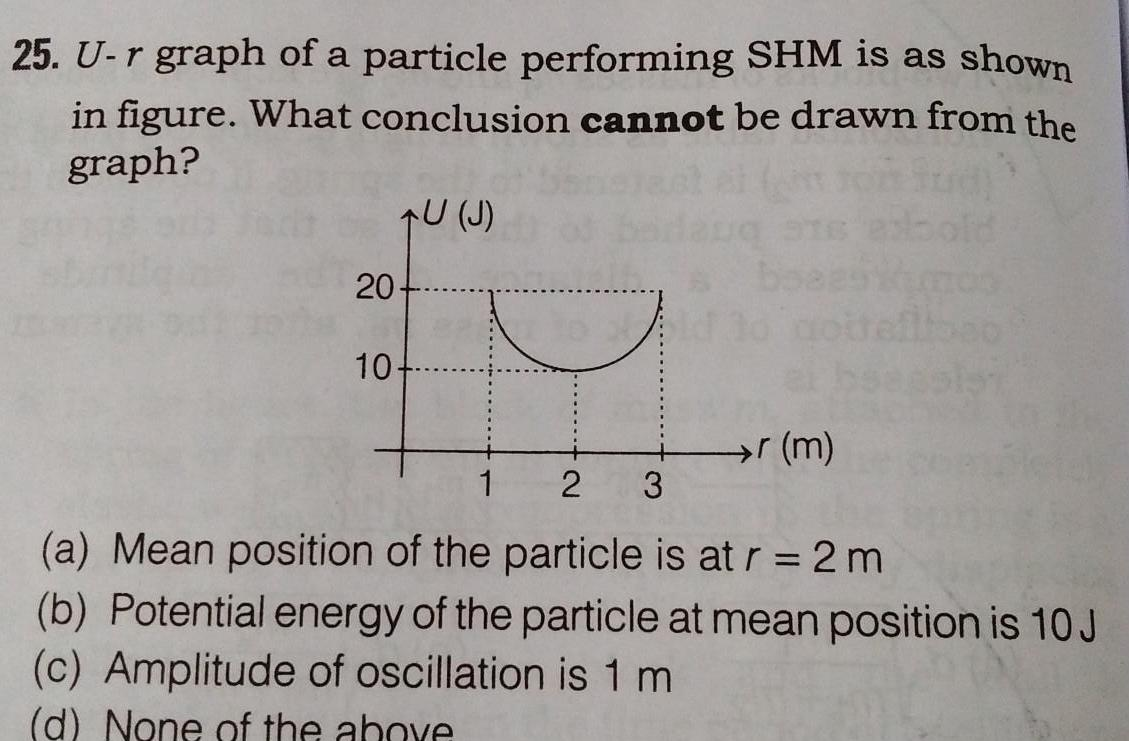Physics
Simple harmonic motion
25 U r graph of a particle performing SHM is as shown in figure What conclusion cannot be drawn from the graph 20 10 U J r m 1 2 3 a Mean position of the particle is at r 2m b Potential energy of the particle at mean position is 10 J c Amplitude of oscillation is 1 m d None of the above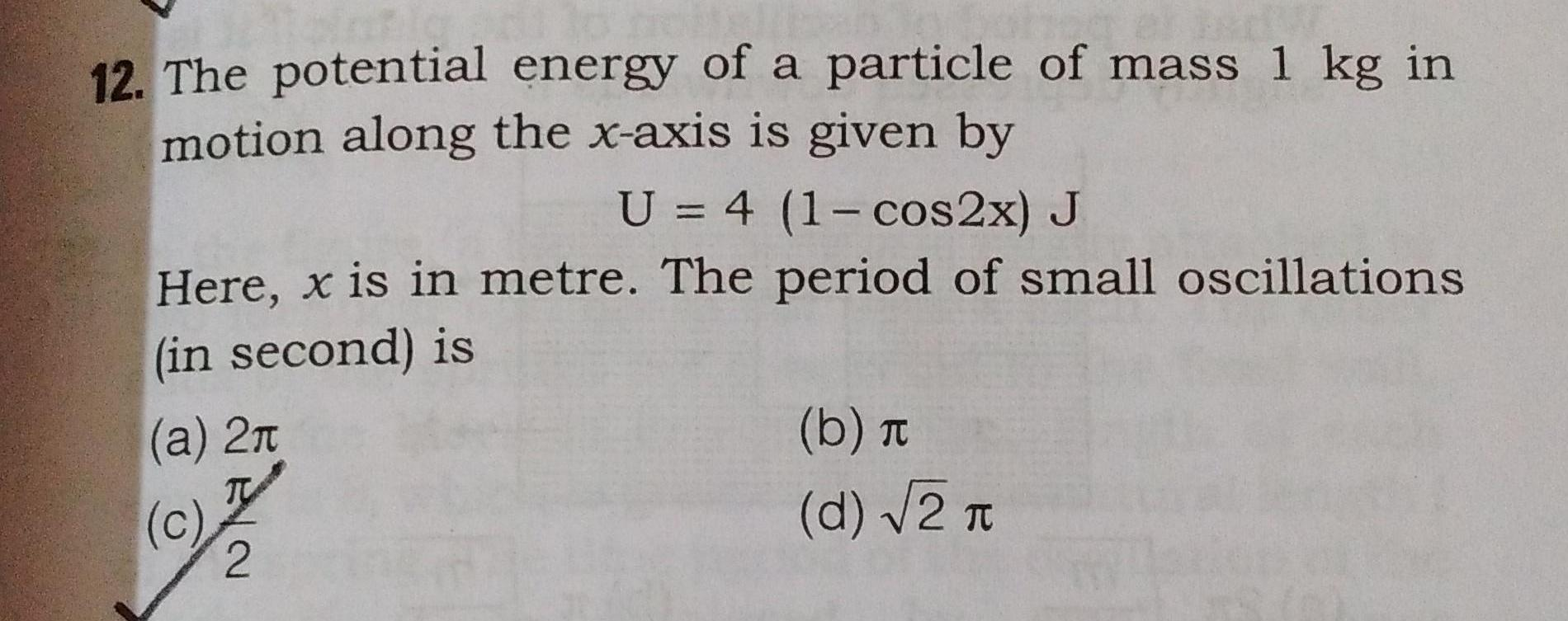Physics
Simple harmonic motion
12 The potential energy of a particle of mass 1 kg in motion along the x axis is given by U 4 1 cos2x J Here x is in metre The period of small oscillations in second is a 2 TV c 2 b d 2 T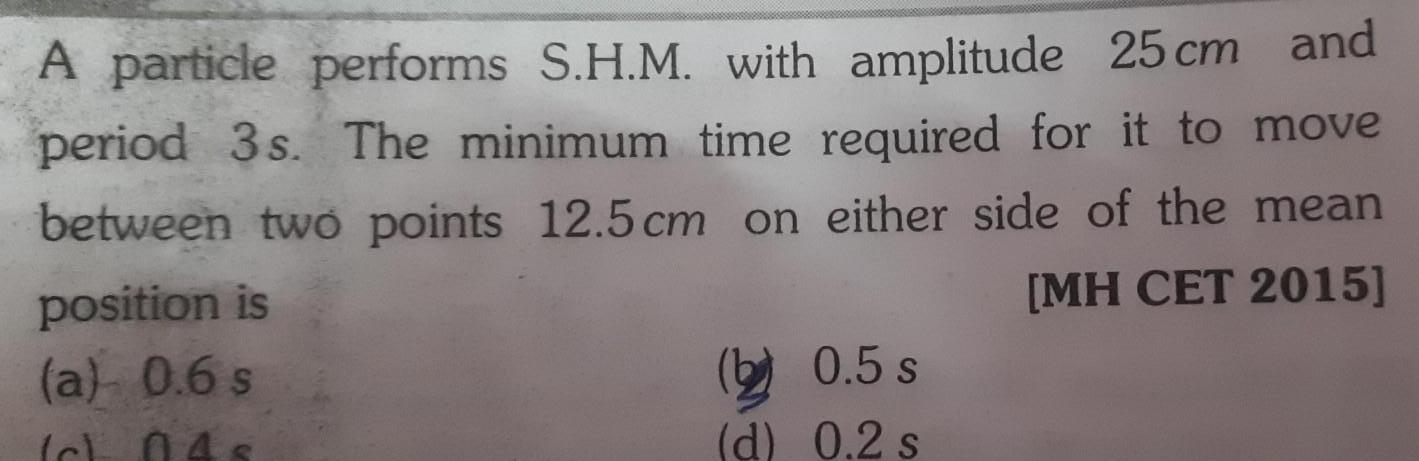Physics
Simple harmonic motion
A particle performs S H M with amplitude 25 cm and period 3s The minimum time required for it to move between two points 12 5 cm on either side of the mean MH CET 2015 position is a 0 6 s Ich 048 0 5 s d 0 2 s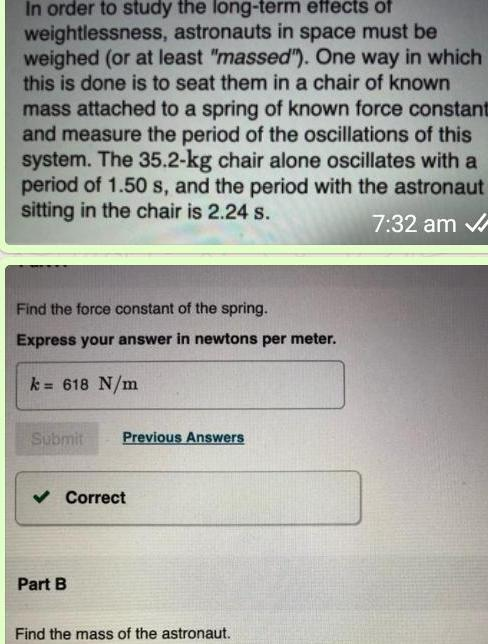Physics
Simple harmonic motion
In order to study the long term effects of weightlessness astronauts in space must be weighed or at least massed One way in which this is done is to seat them in a chair of known mass attached to a spring of known force constant and measure the period of the oscillations of this system The 35 2 kg chair alone oscillates with a period of 1 50 s and the period with the astronaut sitting in the chair is 2 24 s 7 32 am Find the force constant of the spring Express your answer in newtons per meter k 618 N m Submit Previous Answers Correct Part B Find the mass of the astronaut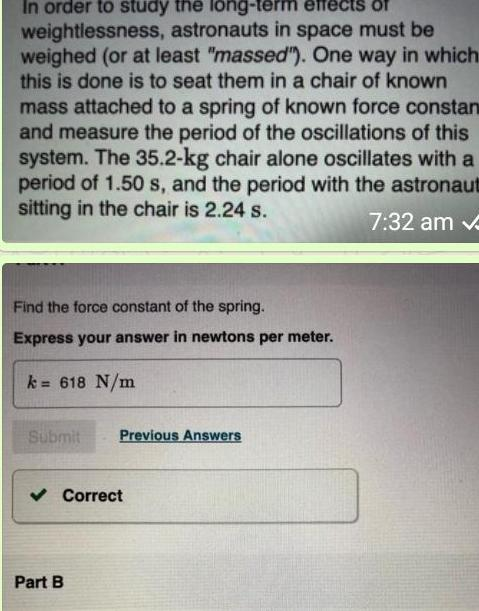Physics
Simple harmonic motion
In order to study the long term effects of weightlessness astronauts in space must be weighed or at least massed One way in which this is done is to seat them in a chair of known mass attached to a spring of known force constan and measure the period of the oscillations of this system The 35 2 kg chair alone oscillates with a period of 1 50 s and the period with the astronaut sitting in the chair is 2 24 s 7 32 am v Find the force constant of the spring Express your answer in newtons per meter k 618 N m Submit Previous Answers Correct Part B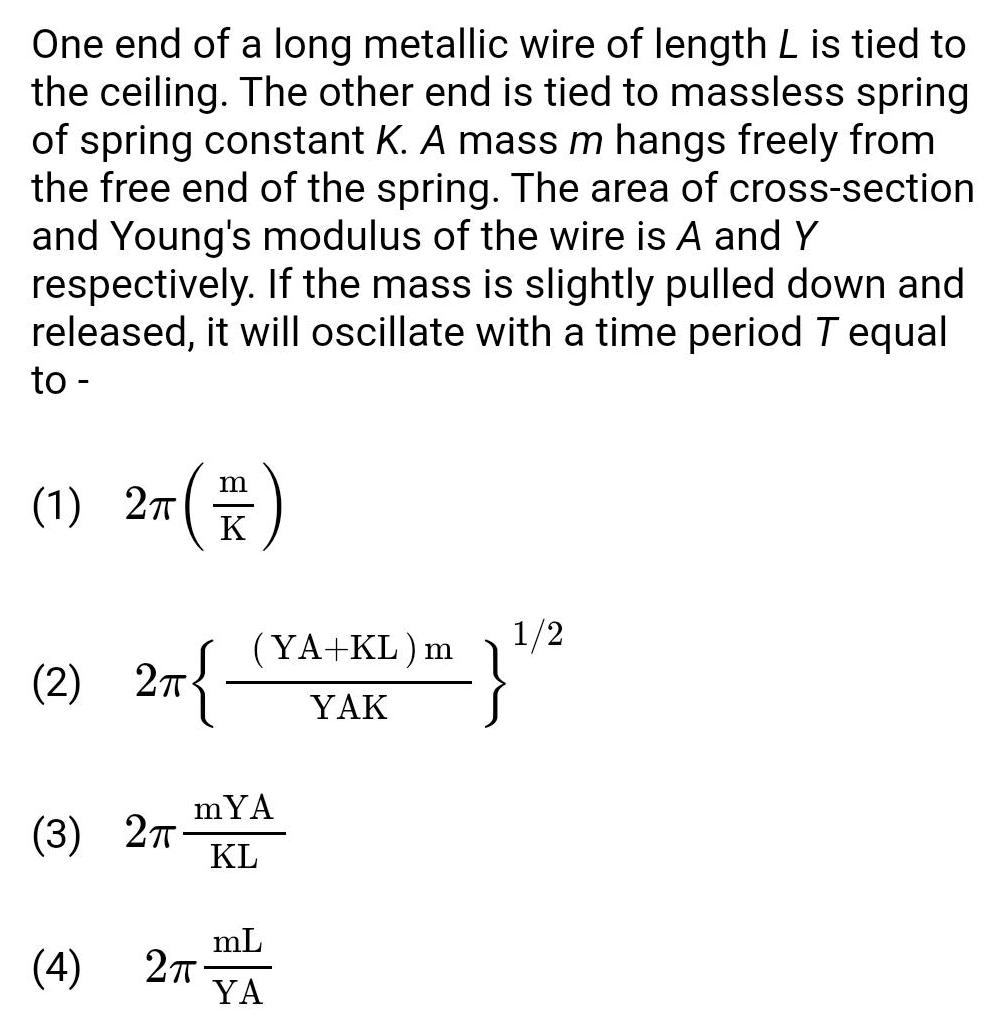Physics
Simple harmonic motion
One end of a long metallic wire of length L is tied to the ceiling The other end is tied to massless spring of spring constant K A mass m hangs freely from the free end of the spring The area of cross section and Young s modulus of the wire is A and Y respectively If the mass is slightly pulled down and released it will oscillate with a time period T equal to 1 2T F 2 2 2 TT 3 2 4 YA KL m YAK mYA KL 2 mL YA 2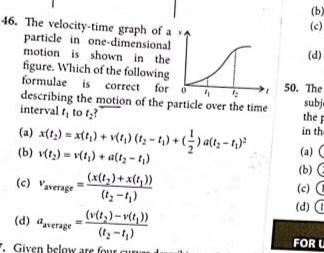Physics
Simple harmonic motion
46 The velocity time graph of a va particle in one dimensional motion is shown in the figure Which of the following formulae is correct for o 4 describing the motion of the particle over the time interval t to t a x t x t v t t t 2 a t 1 b v t v t a t c Vaverage x 1 x t 1 4 d average v t v t t Given below are four cun b c d 50 The subj the F in the a b c C d FOR U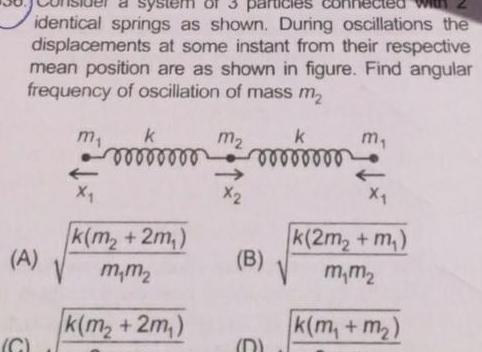Physics
Simple harmonic motion
a system of 3 particles identical springs as shown During oscillations the displacements at some instant from their respective mean position are as shown in figure Find angular frequency of oscillation of mass m A C V m X 00000000 k m 2m m m k m 2m m X k 00000000 B D m X k 2m m m m k m m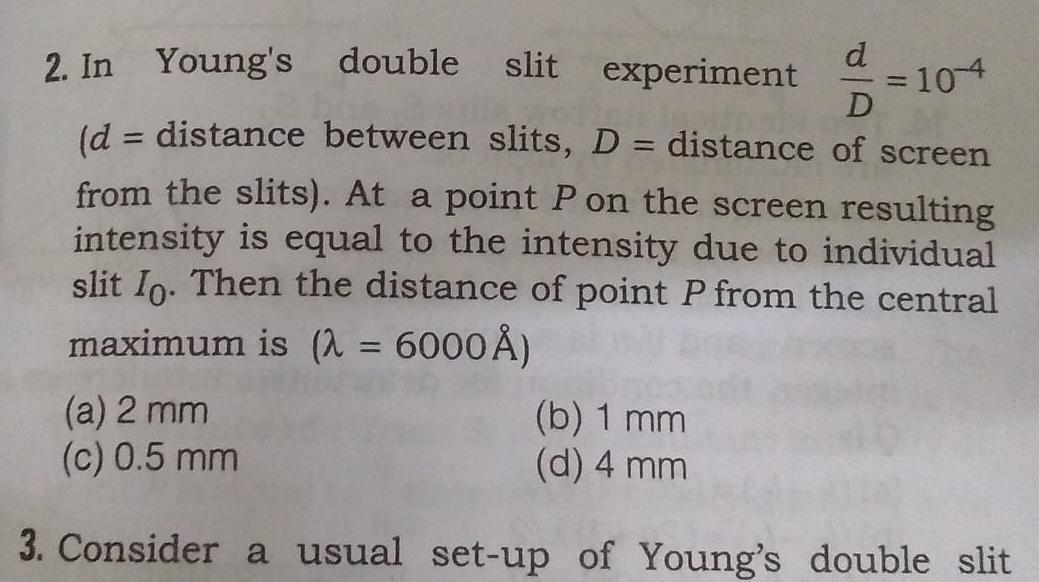Physics
Simple harmonic motion
d 2 In Young s double slit experiment D d distance between slits D distance of screen from the slits At a point P on the screen resulting intensity is equal to the intensity due to individual slit Io Then the distance of point P from the central maximum is 6000 104 a 2 mm b 1 mm d 4 mm c 0 5 mm 3 Consider a usual set up of Young s double slit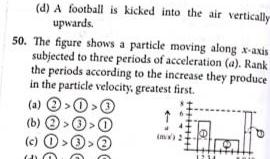Physics
Simple harmonic motion
d A football is kicked into the air vertically upwards 50 The figure shows a particle moving along x axis subjected to three periods of acceleration a Rank the periods according to the increase they produce in the particle velocity greatest first a b c 0 0 0 6 m 2 e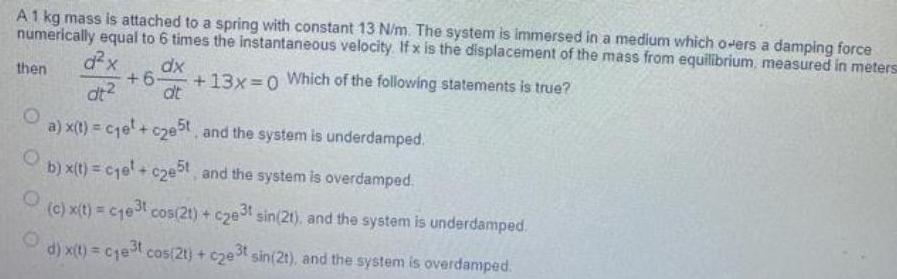Physics
Simple harmonic motion
A 1 kg mass is attached to a spring with constant 13 N m The system is immersed in a medium which o ers a damping force numerically equal to 6 times the instantaneous velocity If x is the displacement of the mass from equilibrium measured in meters d x 6 13x 0 Which of the following statements is true dx dt dt then O a x t c e c e5t and the system is underdamped O b x t c e c2e5t and the system is overdamped O c x t c1e t cos 21 c2e3t sin 21 and the system is underdamped O d x t c e t cos 2t c2e3t sin 2t and the system is overdamped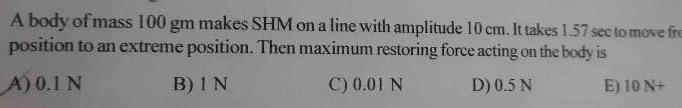Physics
Simple harmonic motion
A body of mass 100 gm makes SHM on a line with amplitude 10 cm It takes 1 57 sec to move fre position to an extreme position Then maximum restoring force acting on the body is A 0 1 N B IN C 0 01 N D 0 5 N E 10 N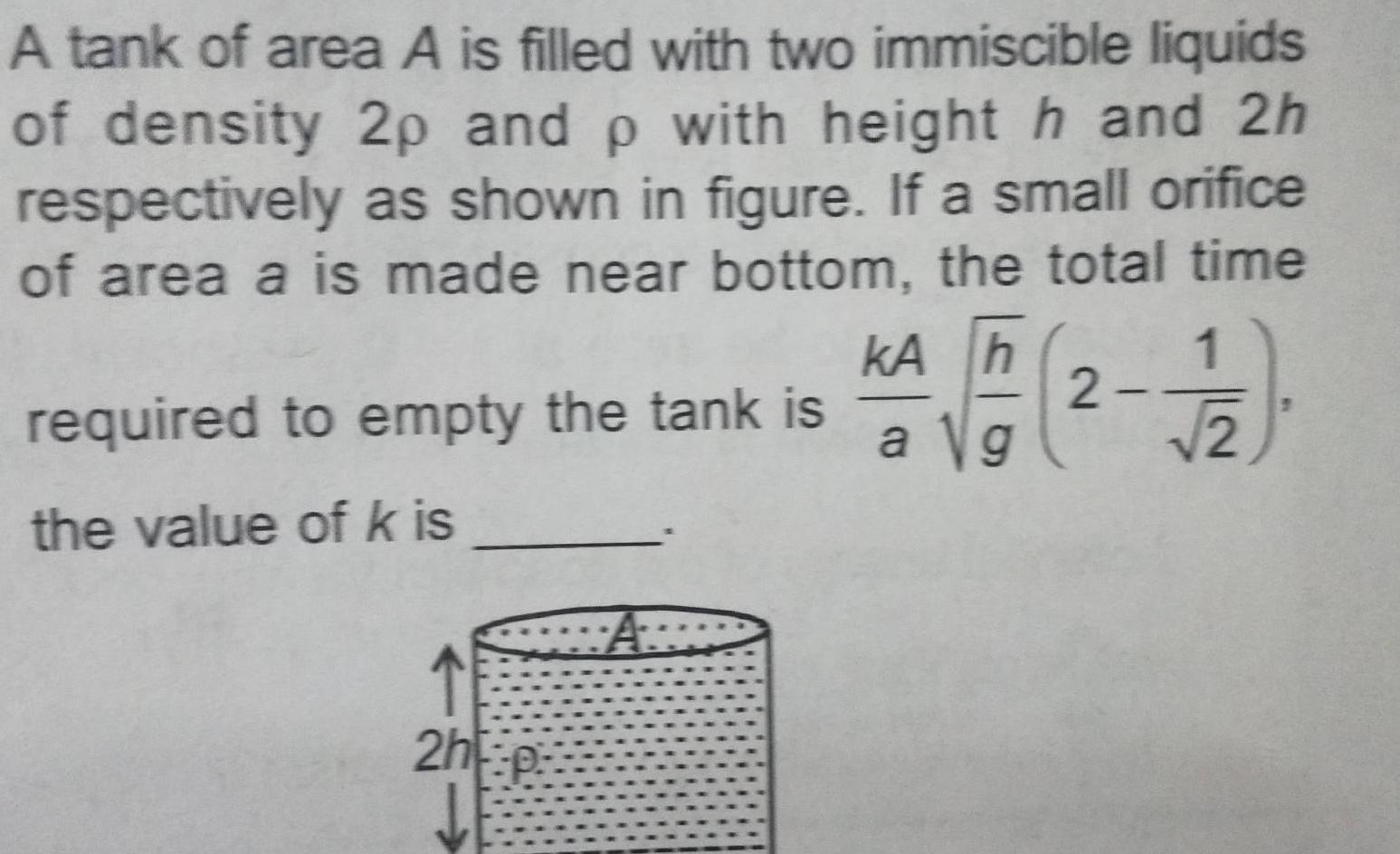Physics
Simple harmonic motion
A tank of area A is filled with two immiscible liquids of density 2p and p with height h and 2h respectively as shown in figure If a small orifice of area a is made near bottom the total time kA h a Vg required to empty the tank is the value of k is 2hp 2 2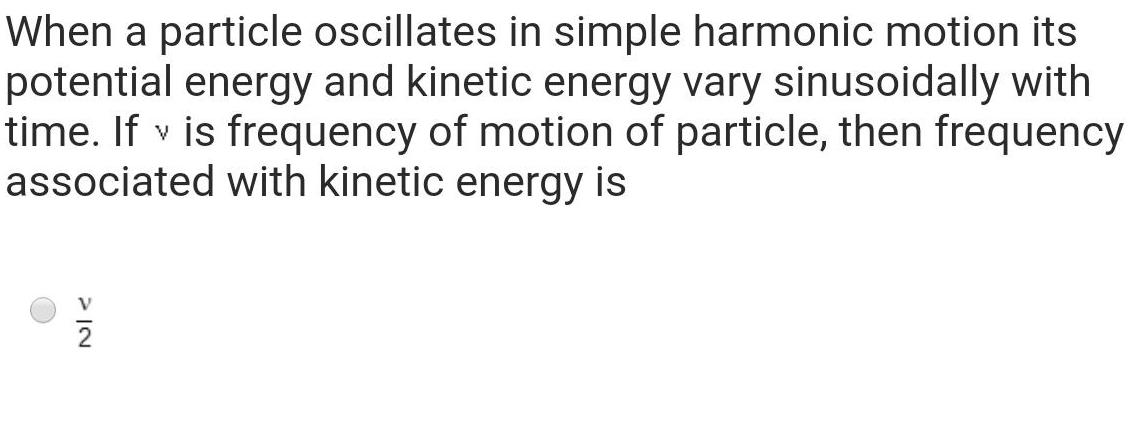Physics
Simple harmonic motion
When a particle oscillates in simple harmonic motion its potential energy and kinetic energy vary sinusoidally with time If v is frequency of motion of particle then frequency associated with kinetic energy is NIC 2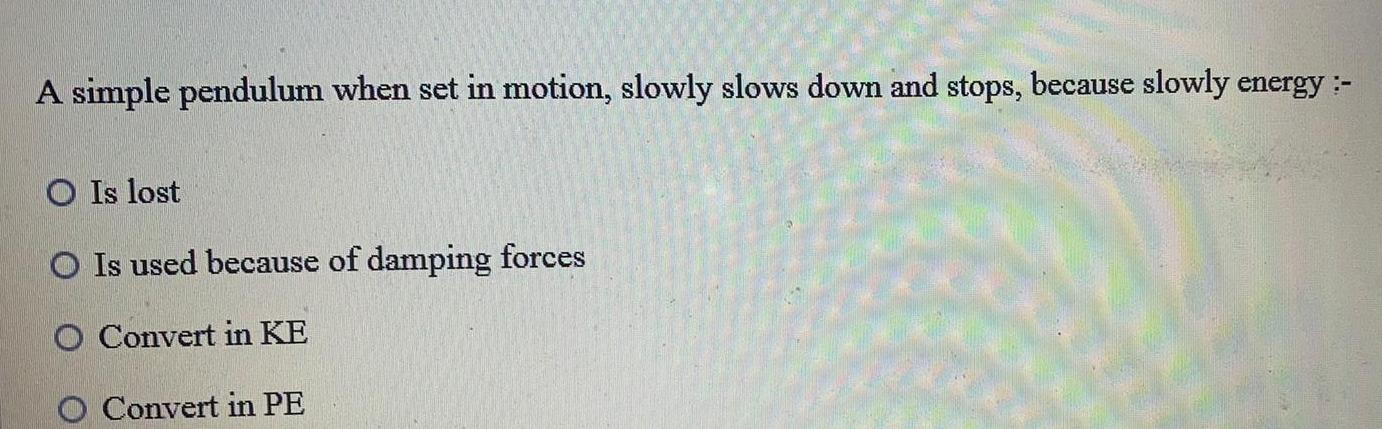Physics
Simple harmonic motion
A simple pendulum when set in motion slowly slows down and stops because slowly energy O Is lost O Is used because of damping forces O Convert in KE Convert in PE